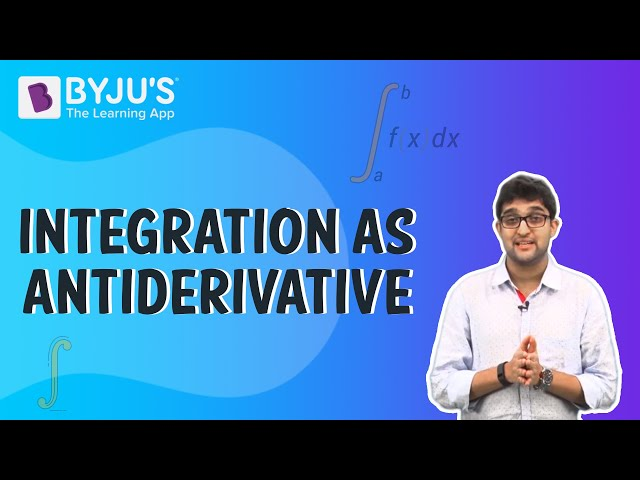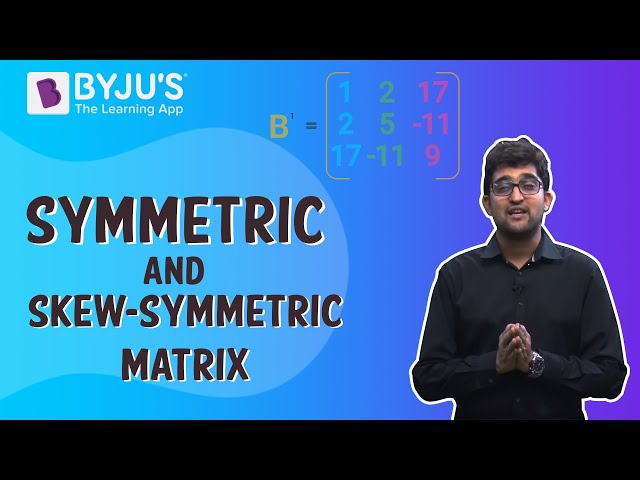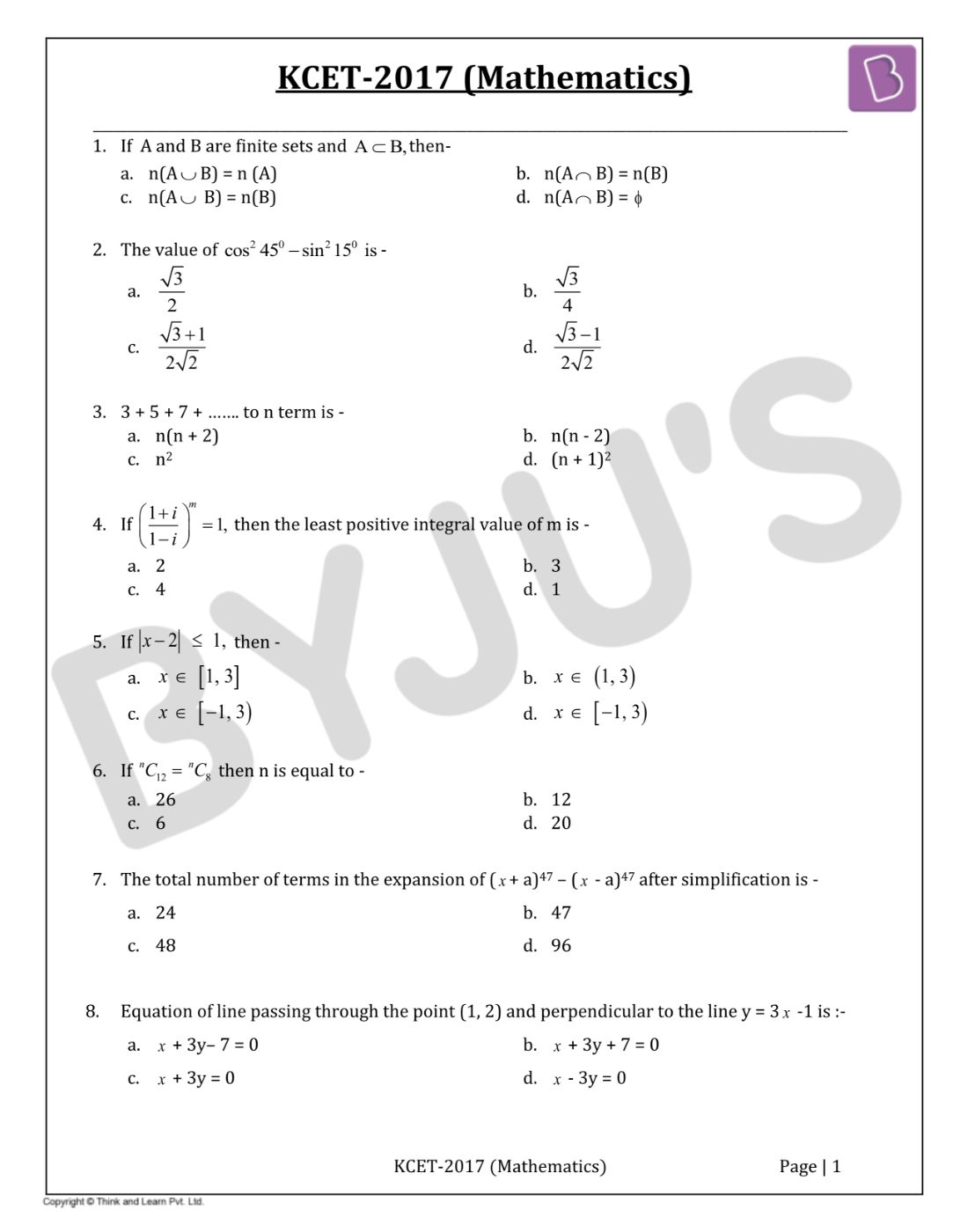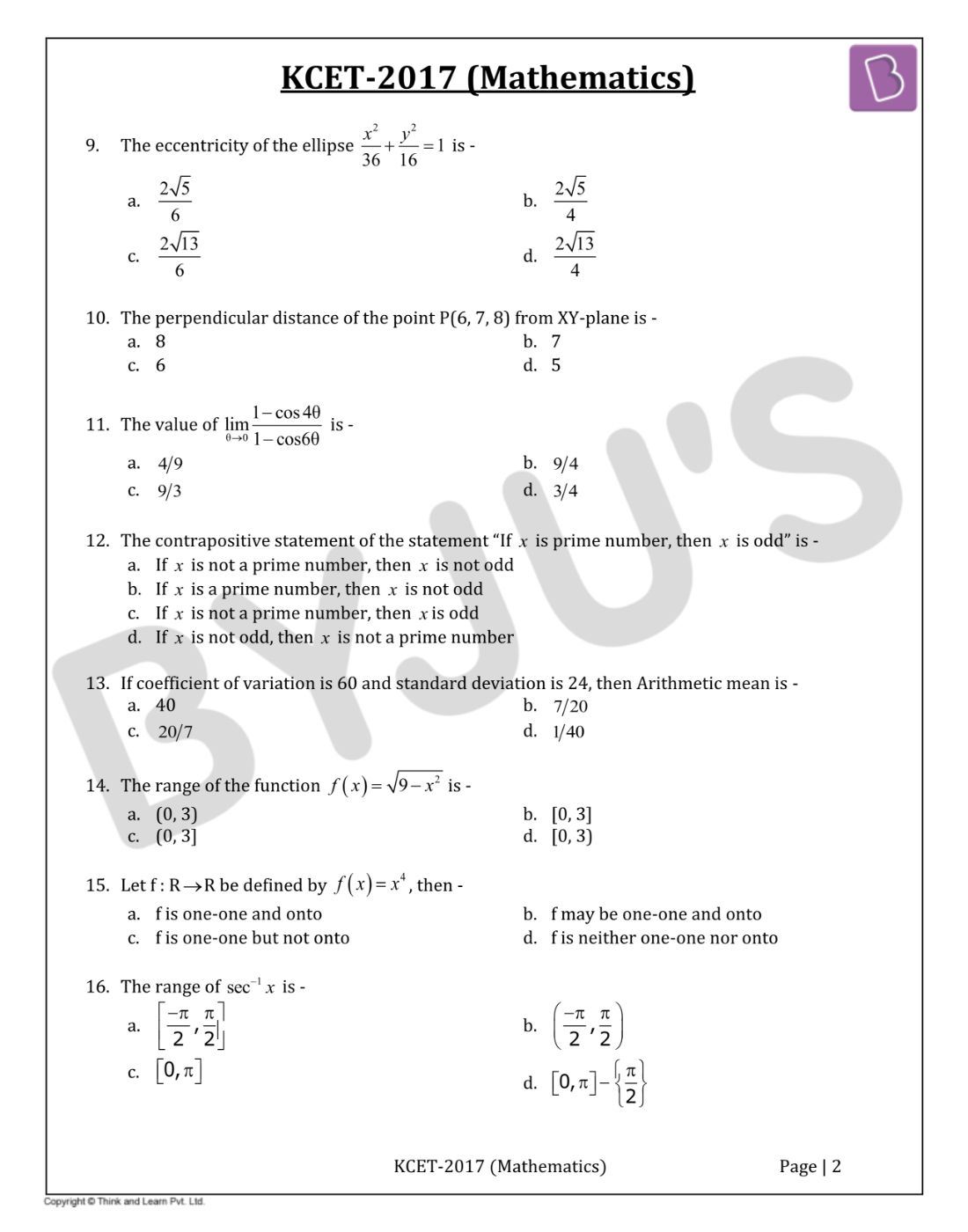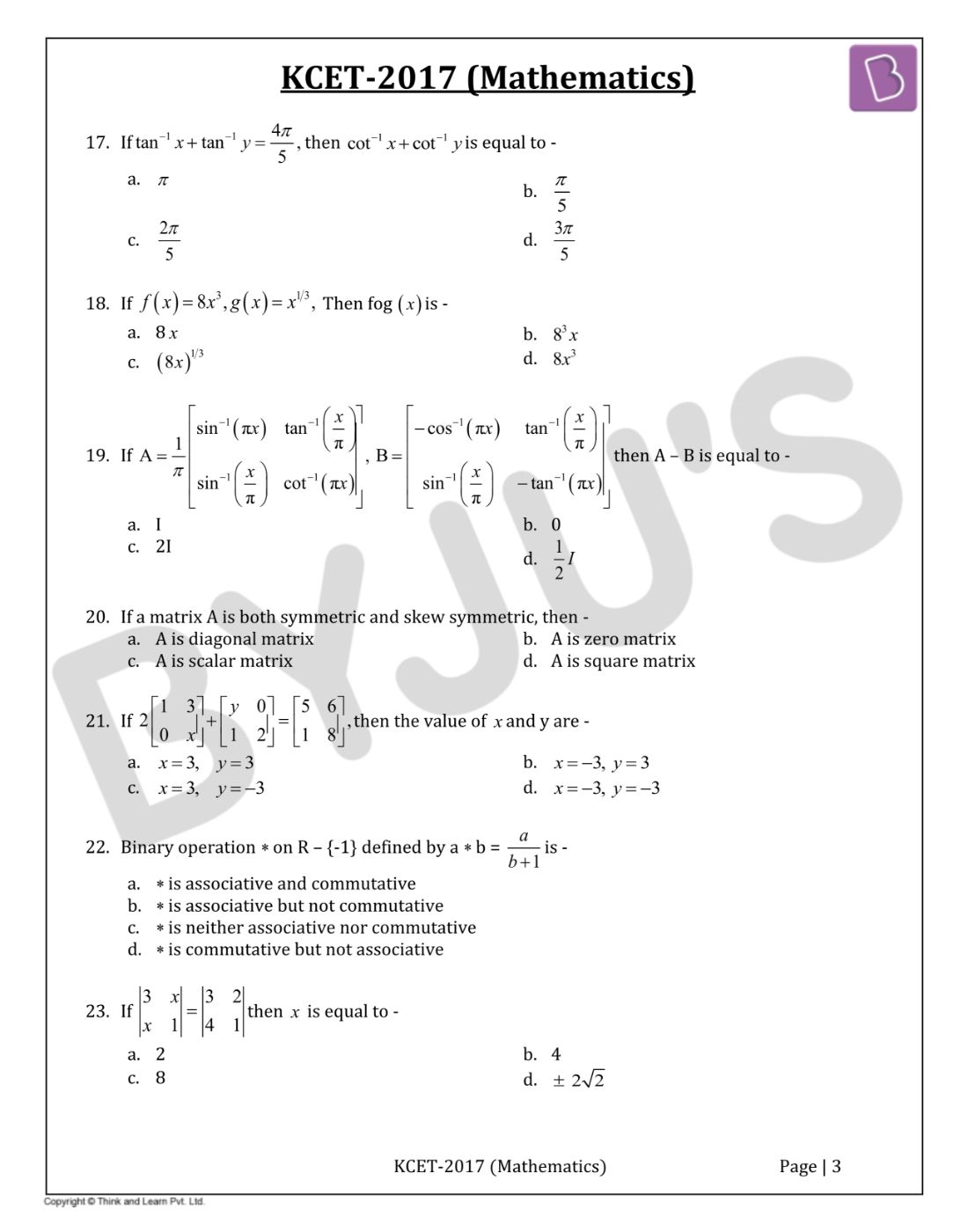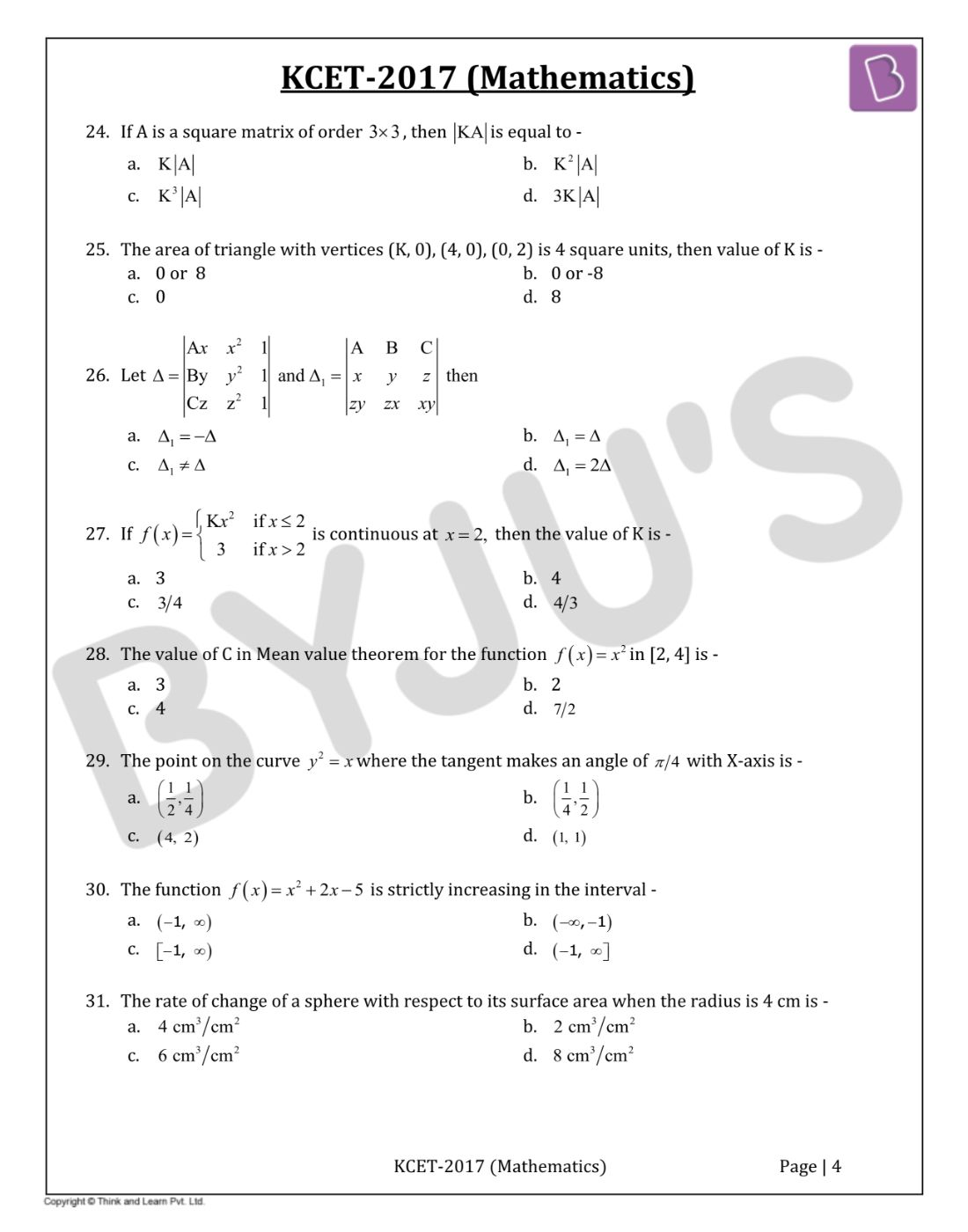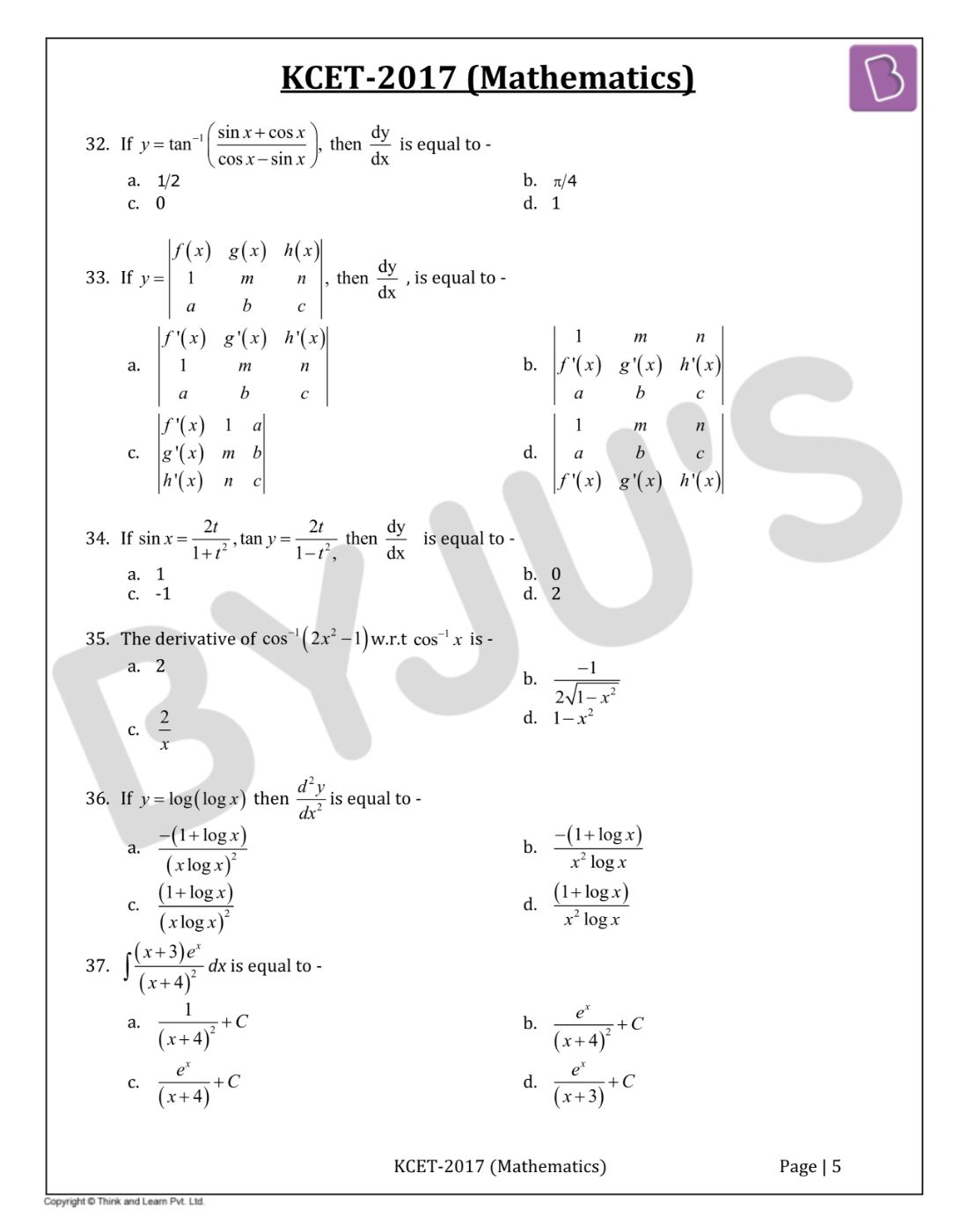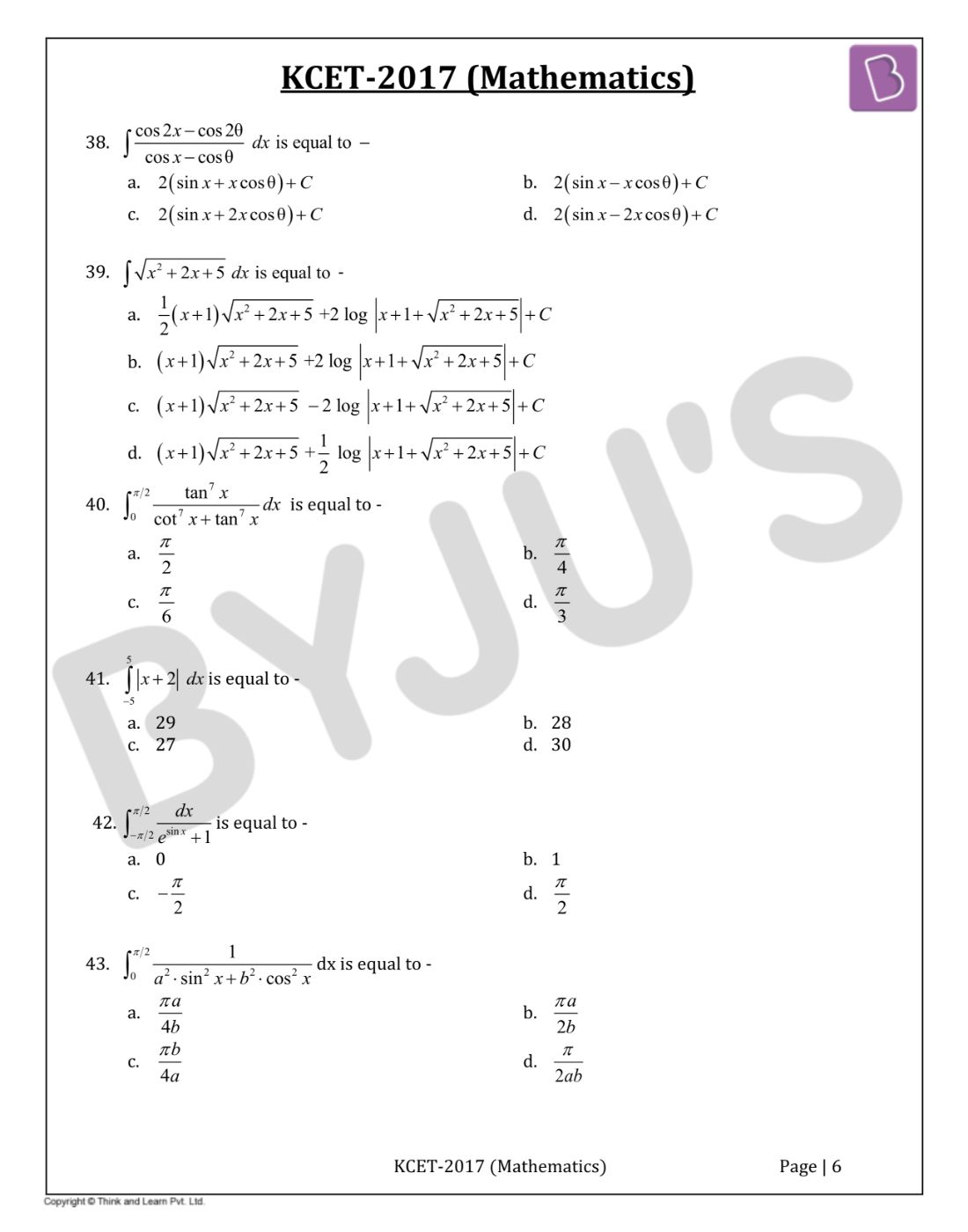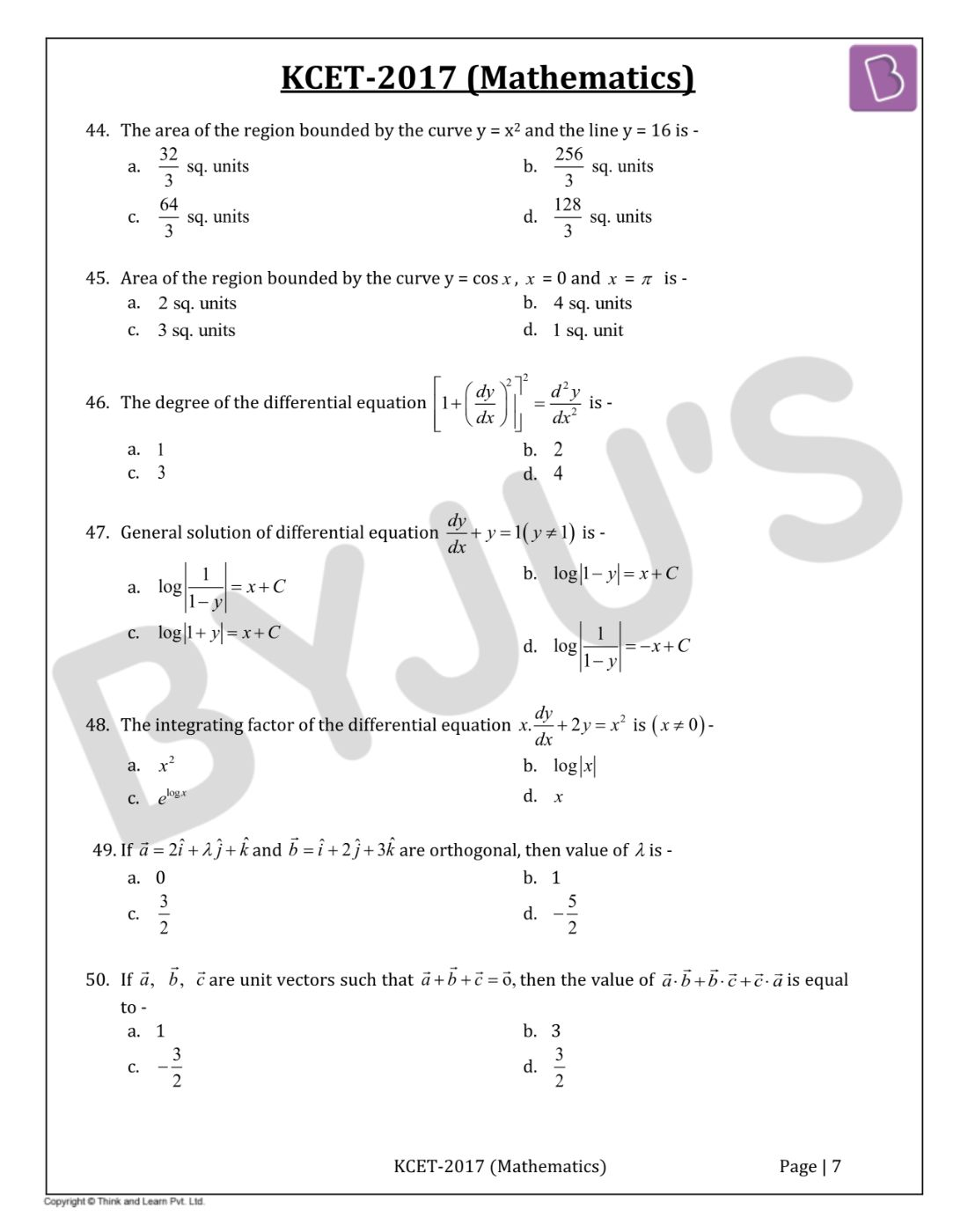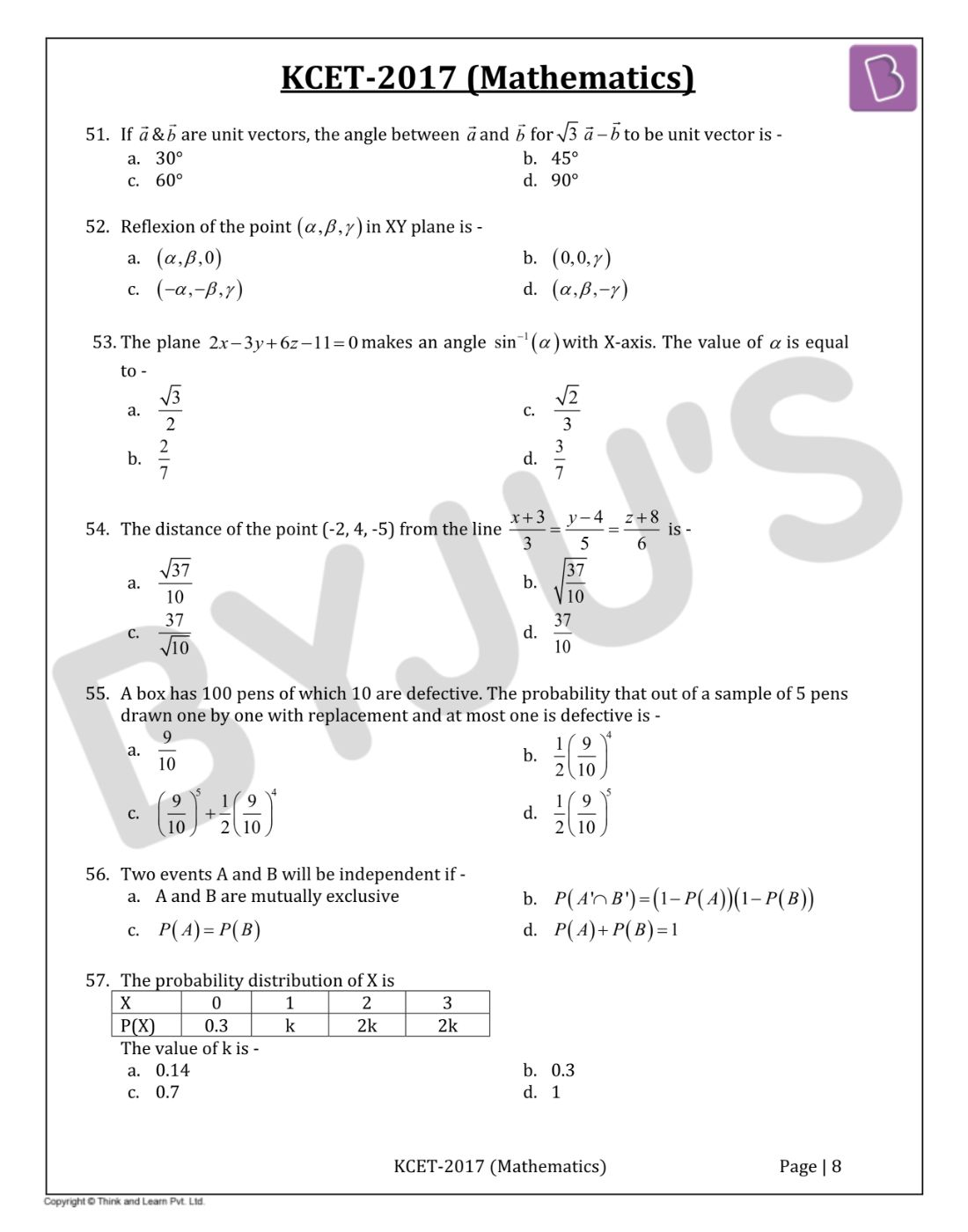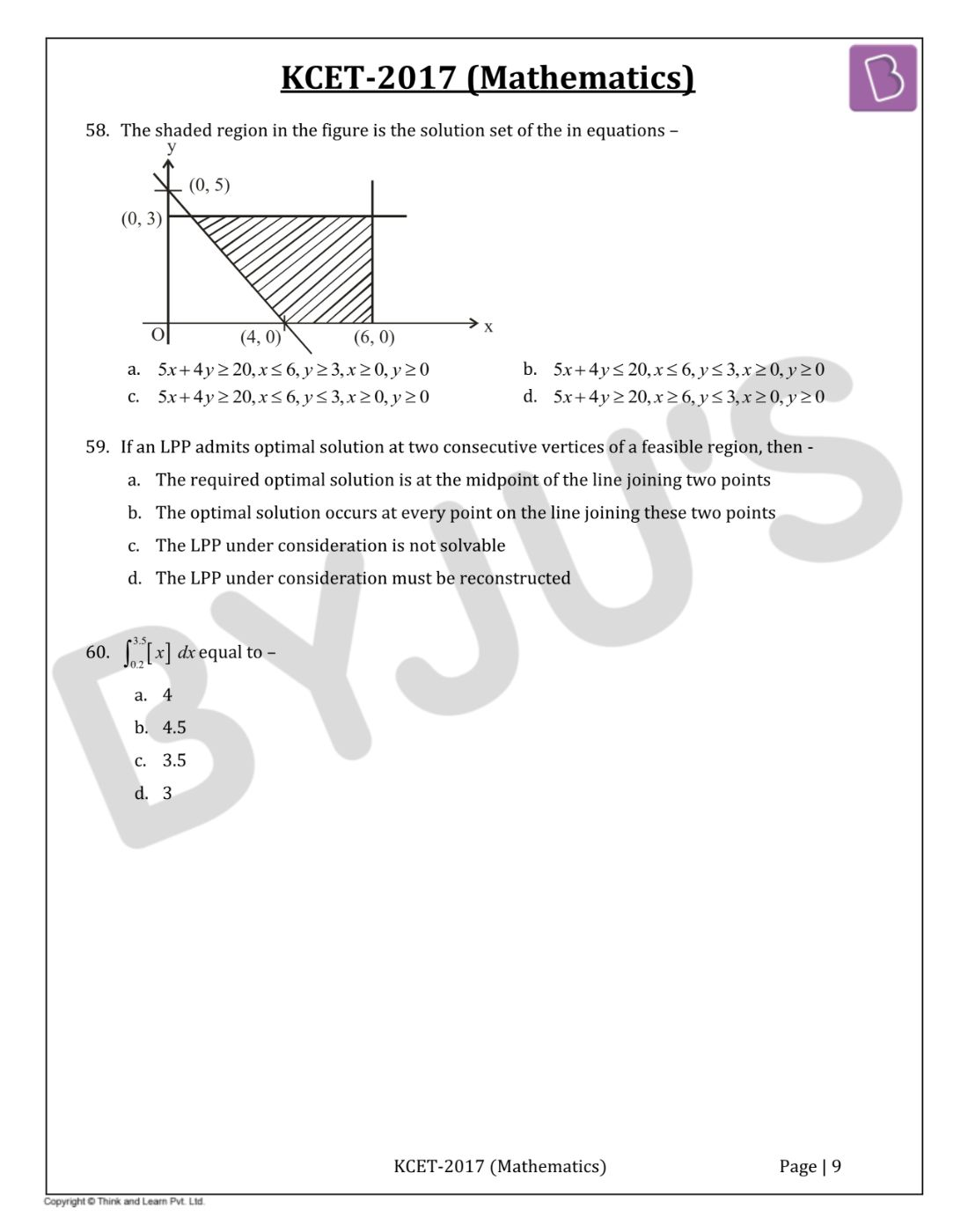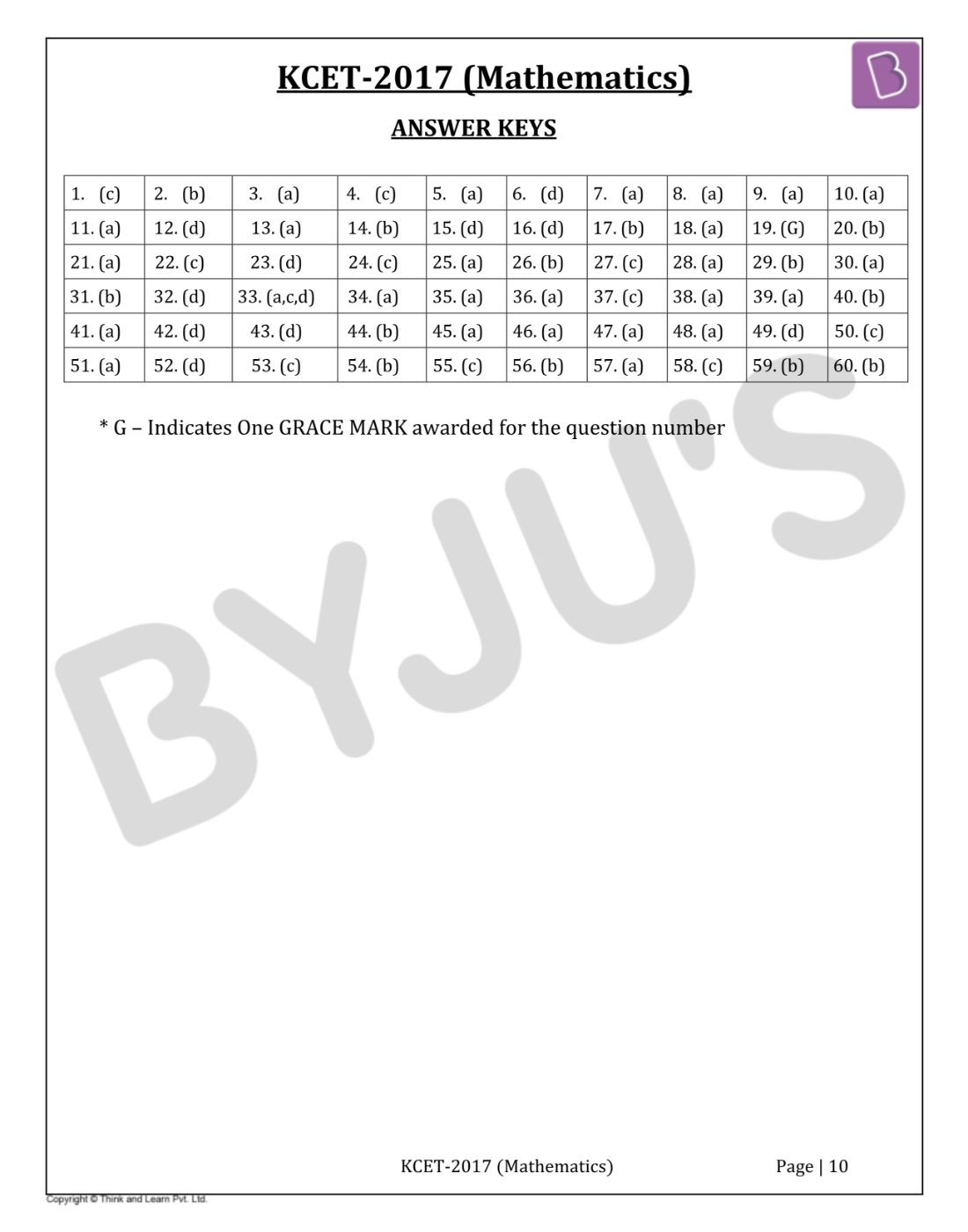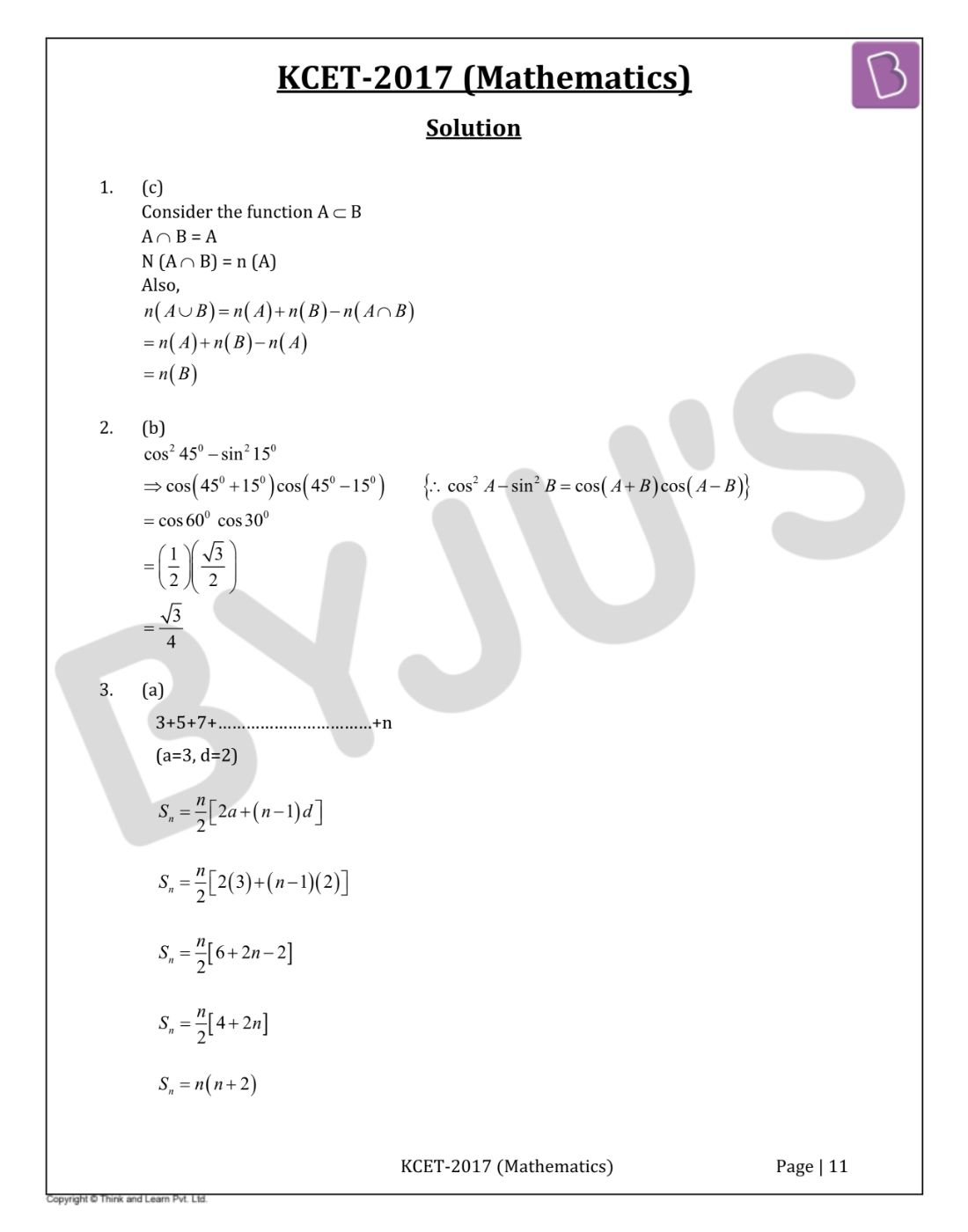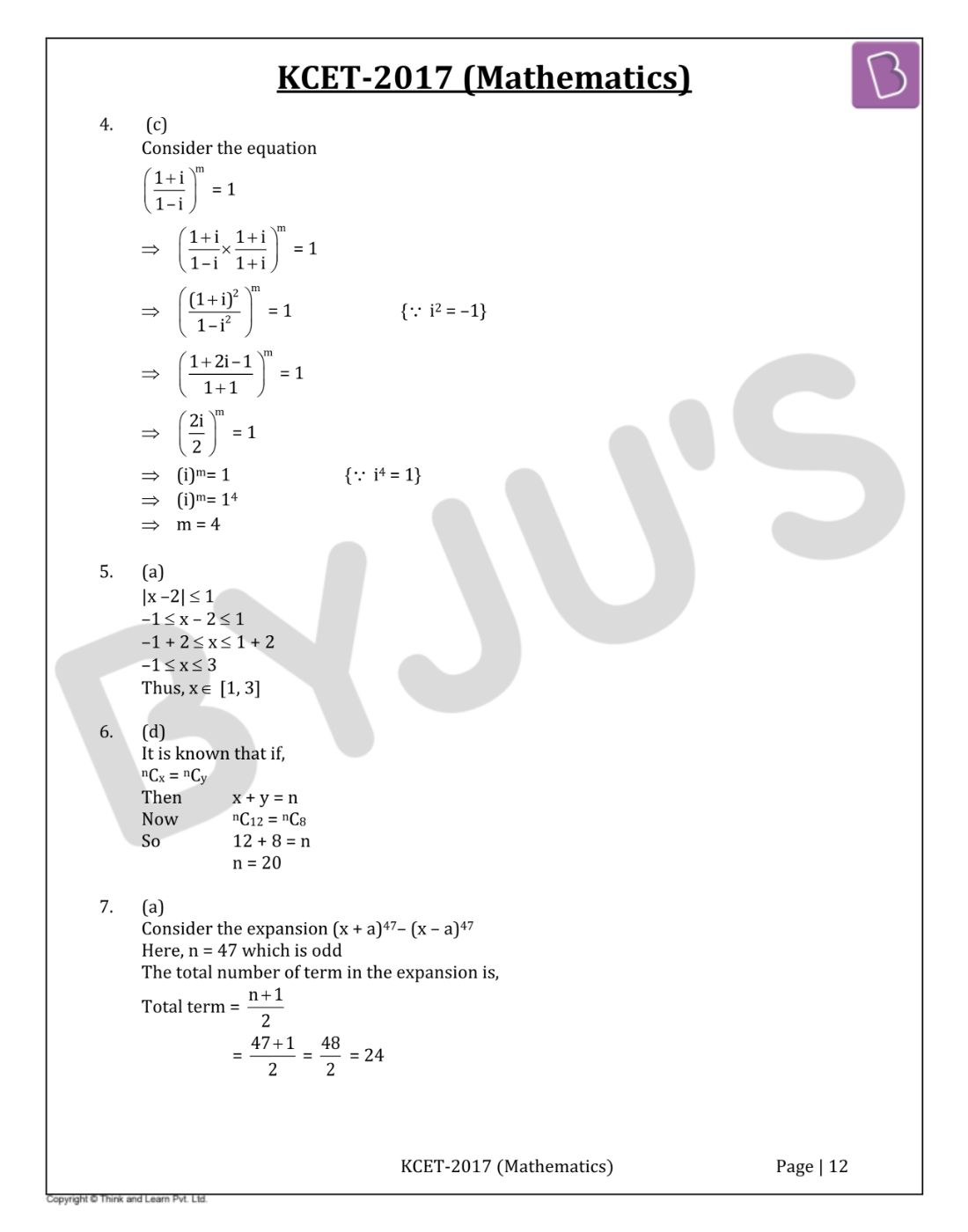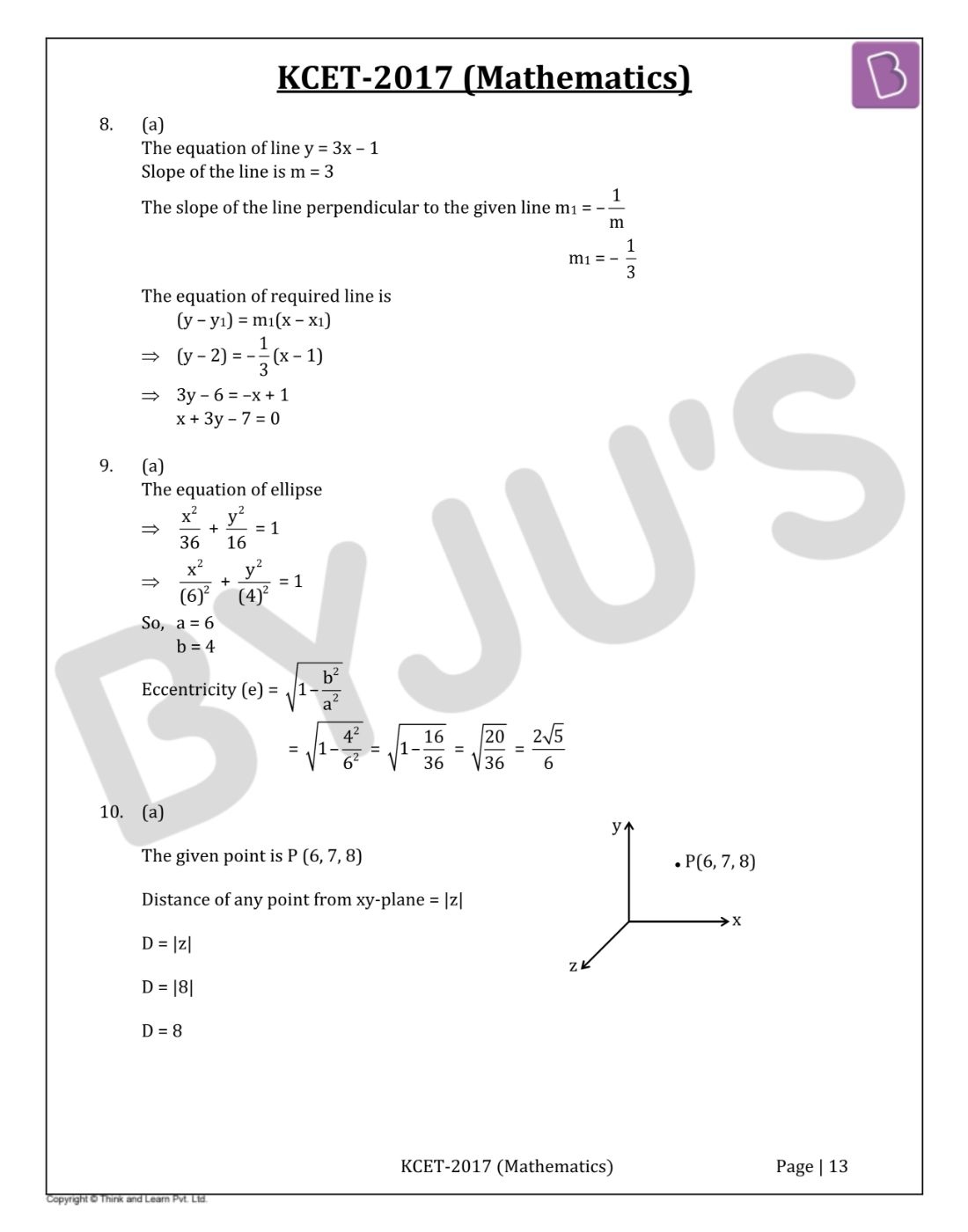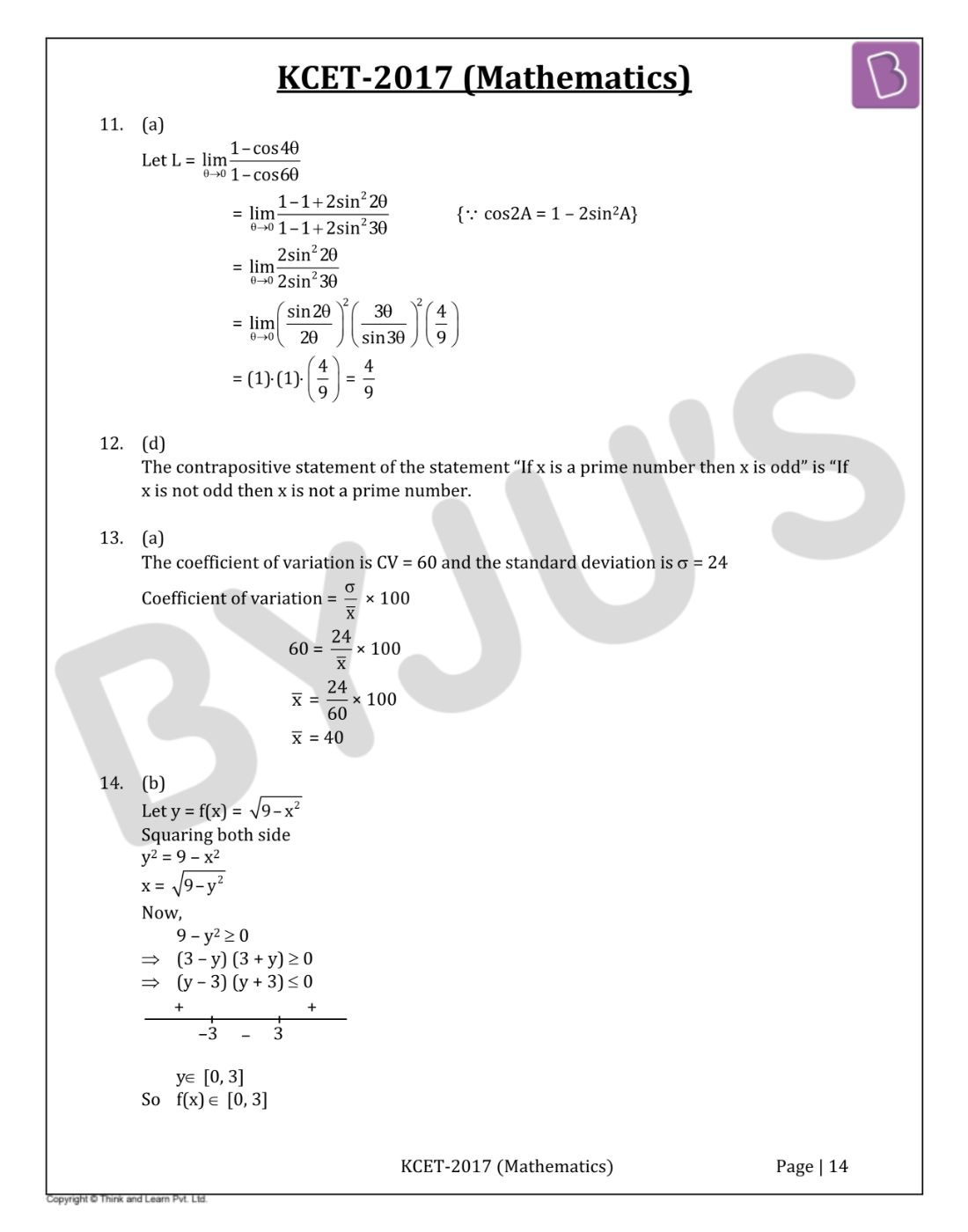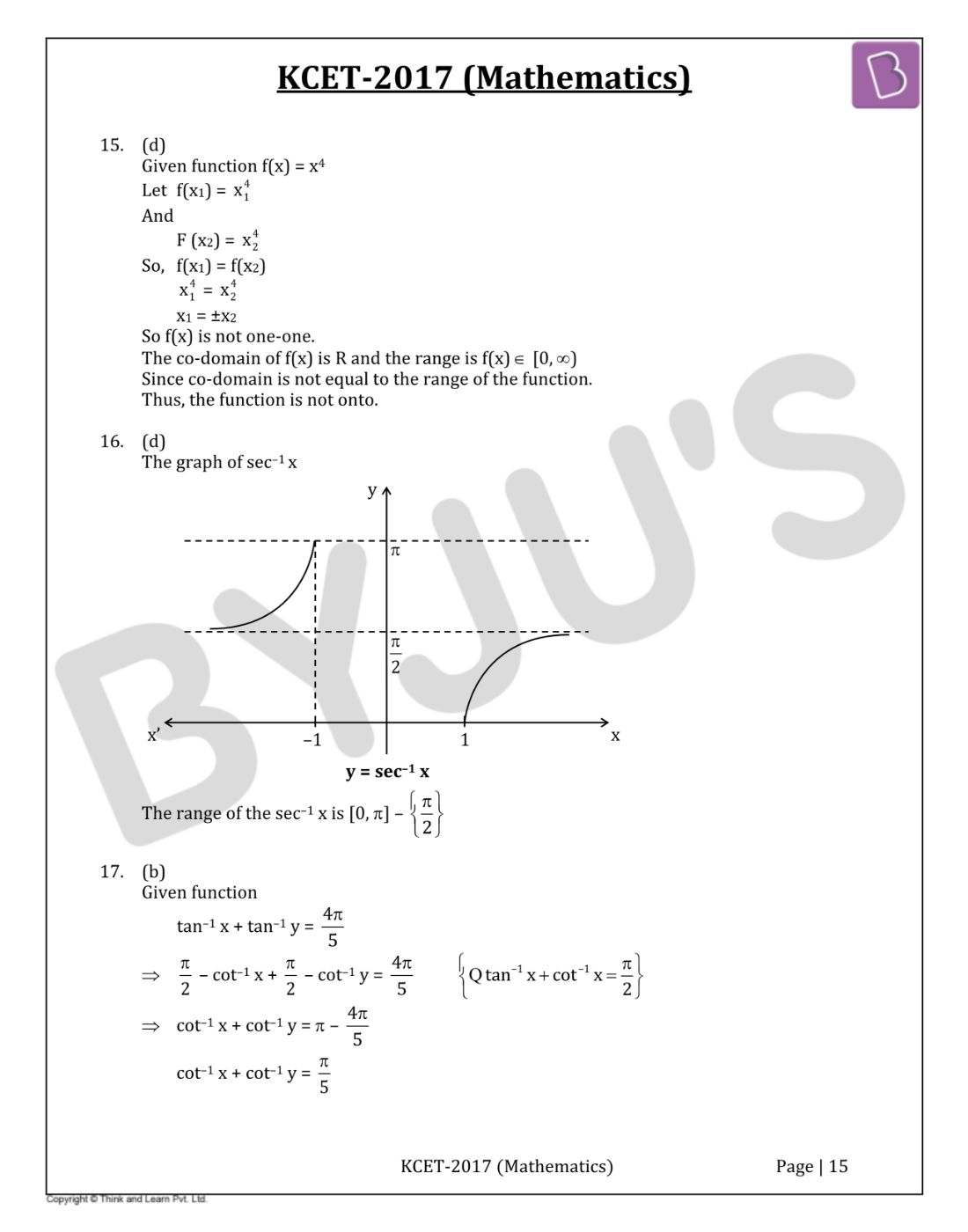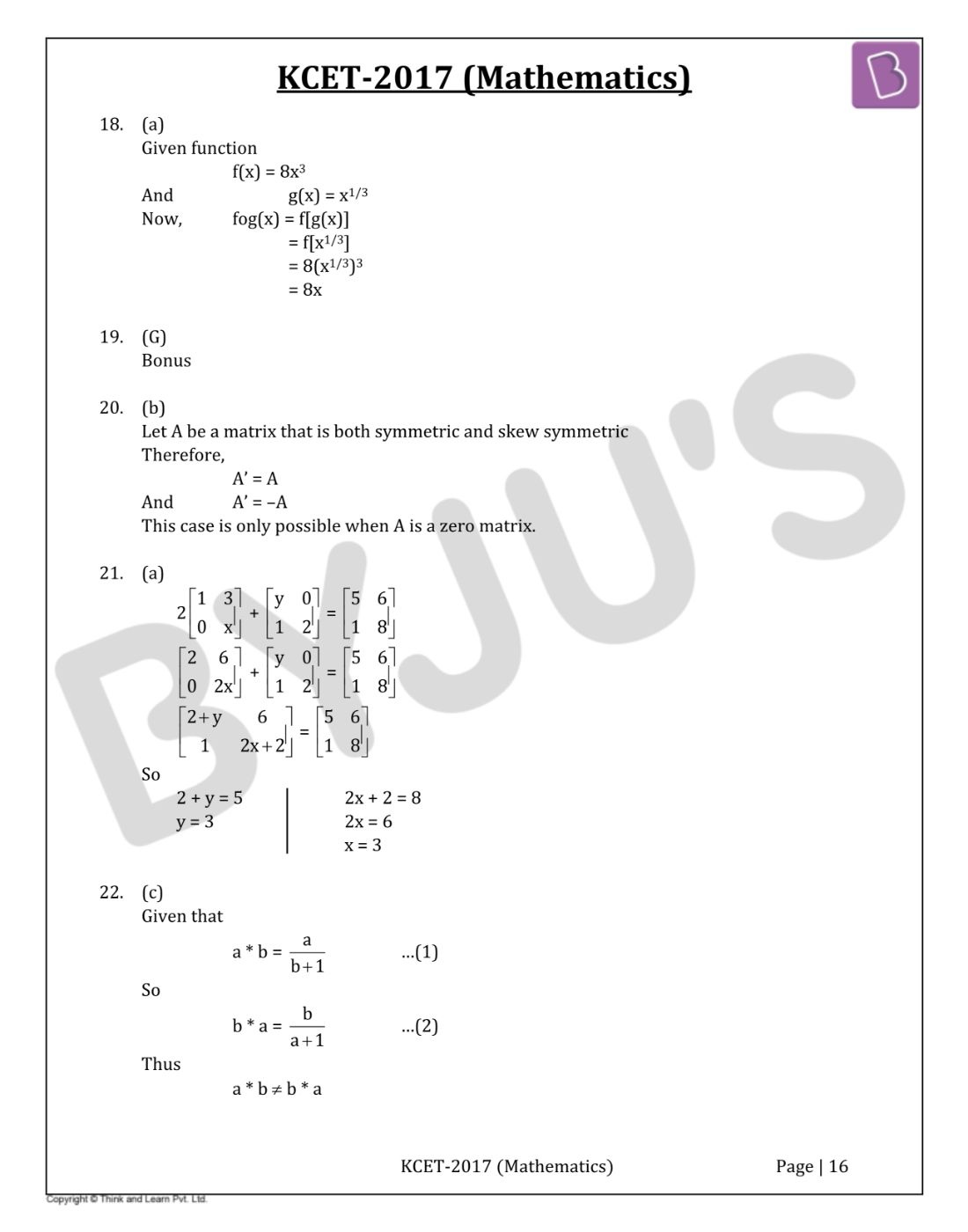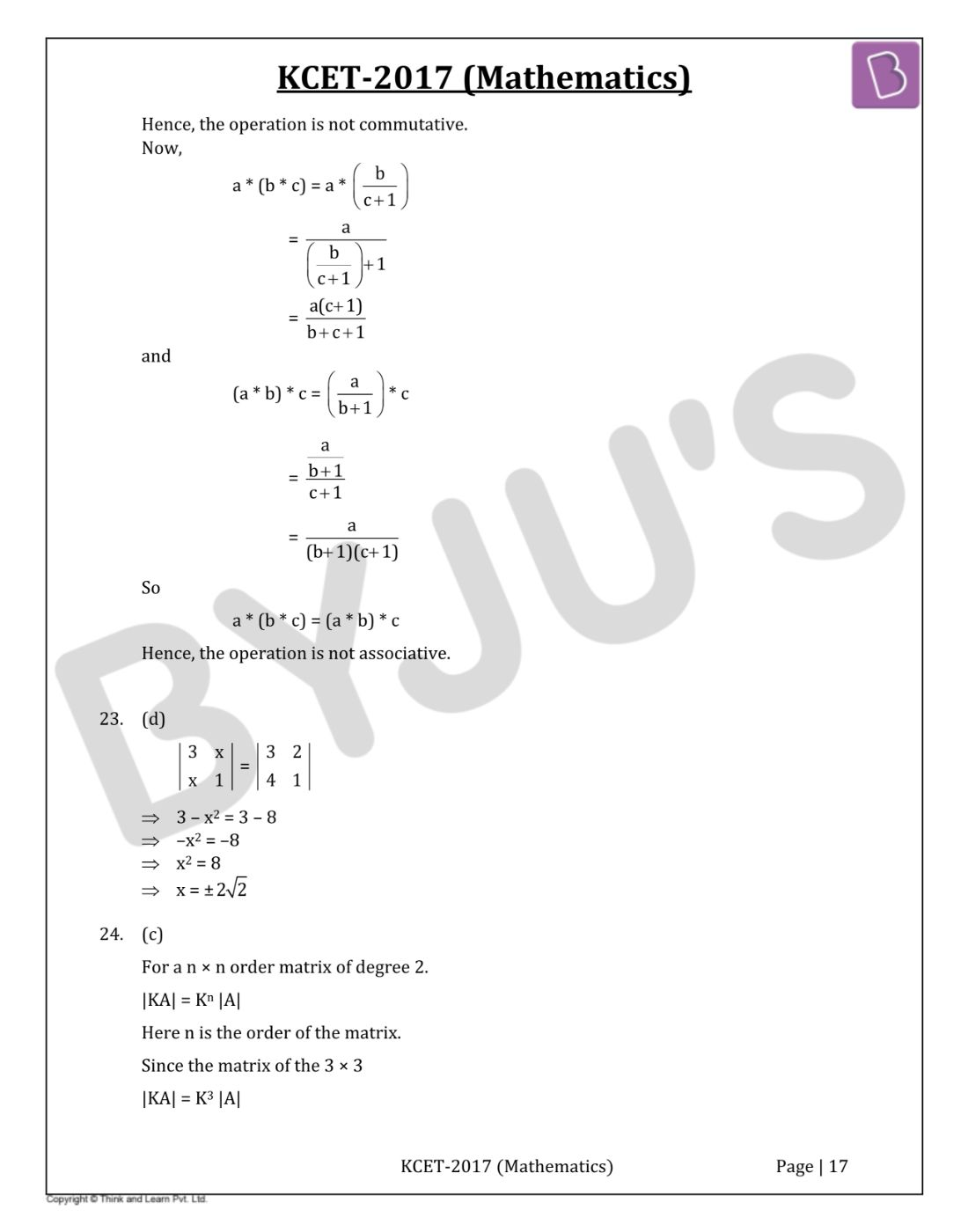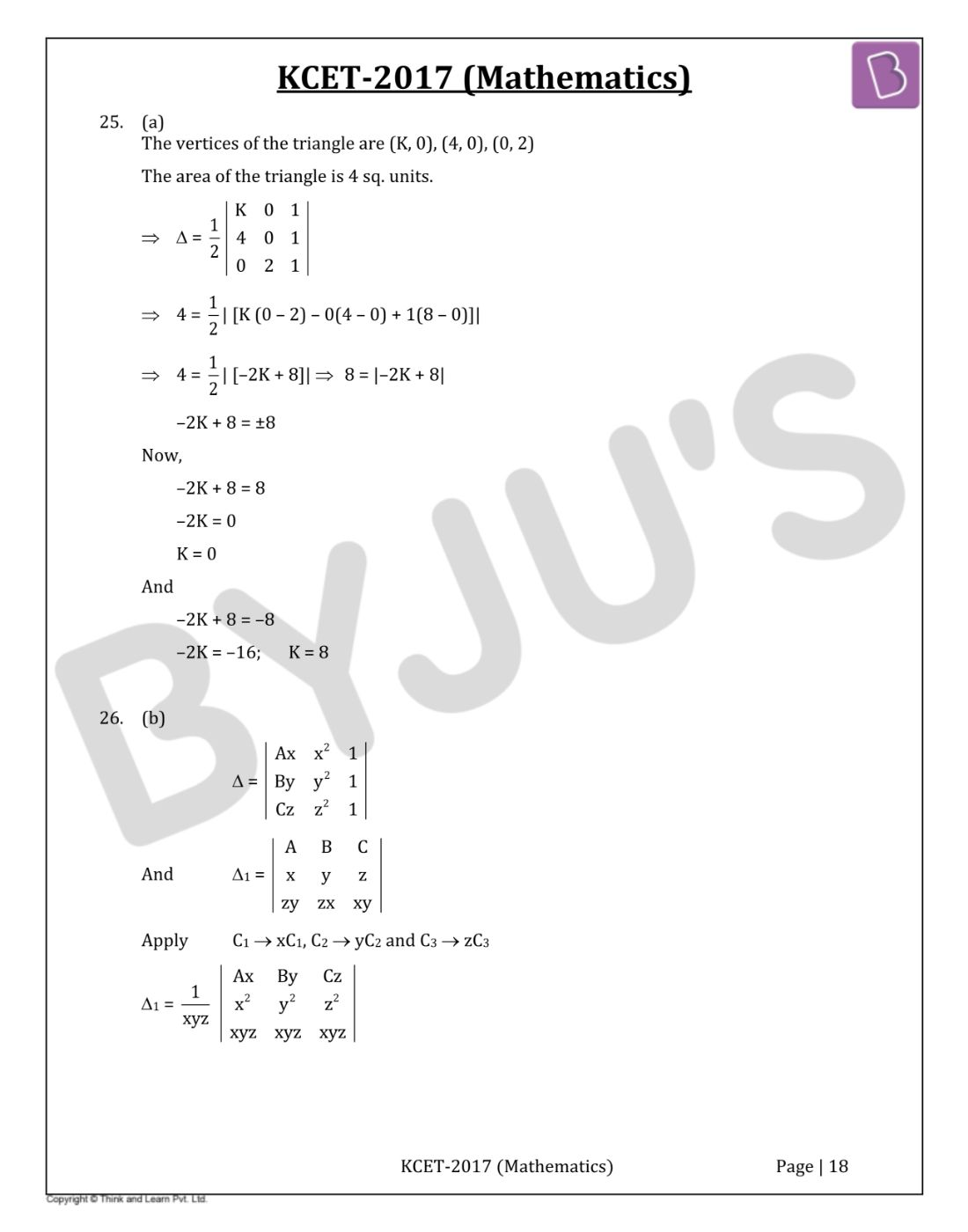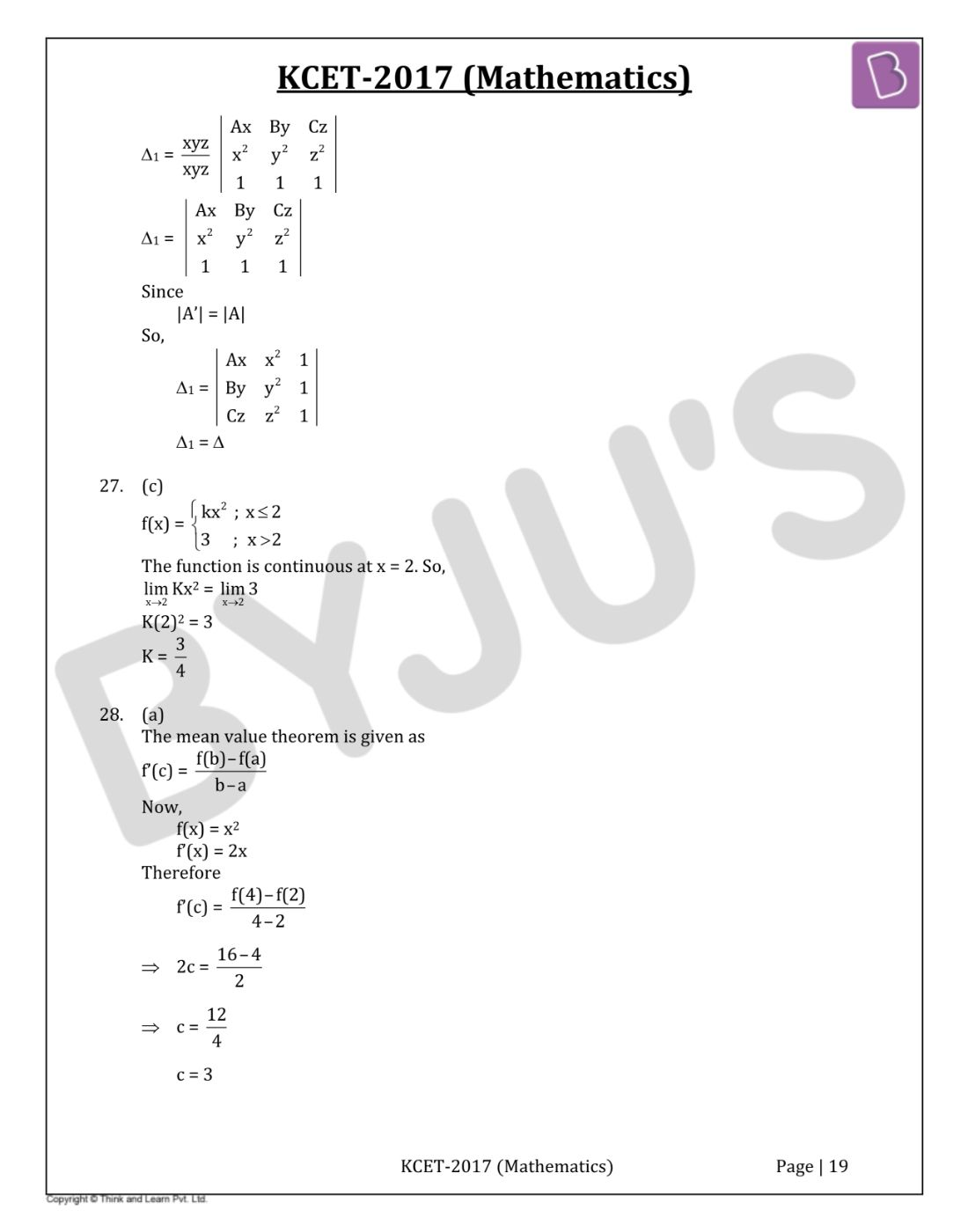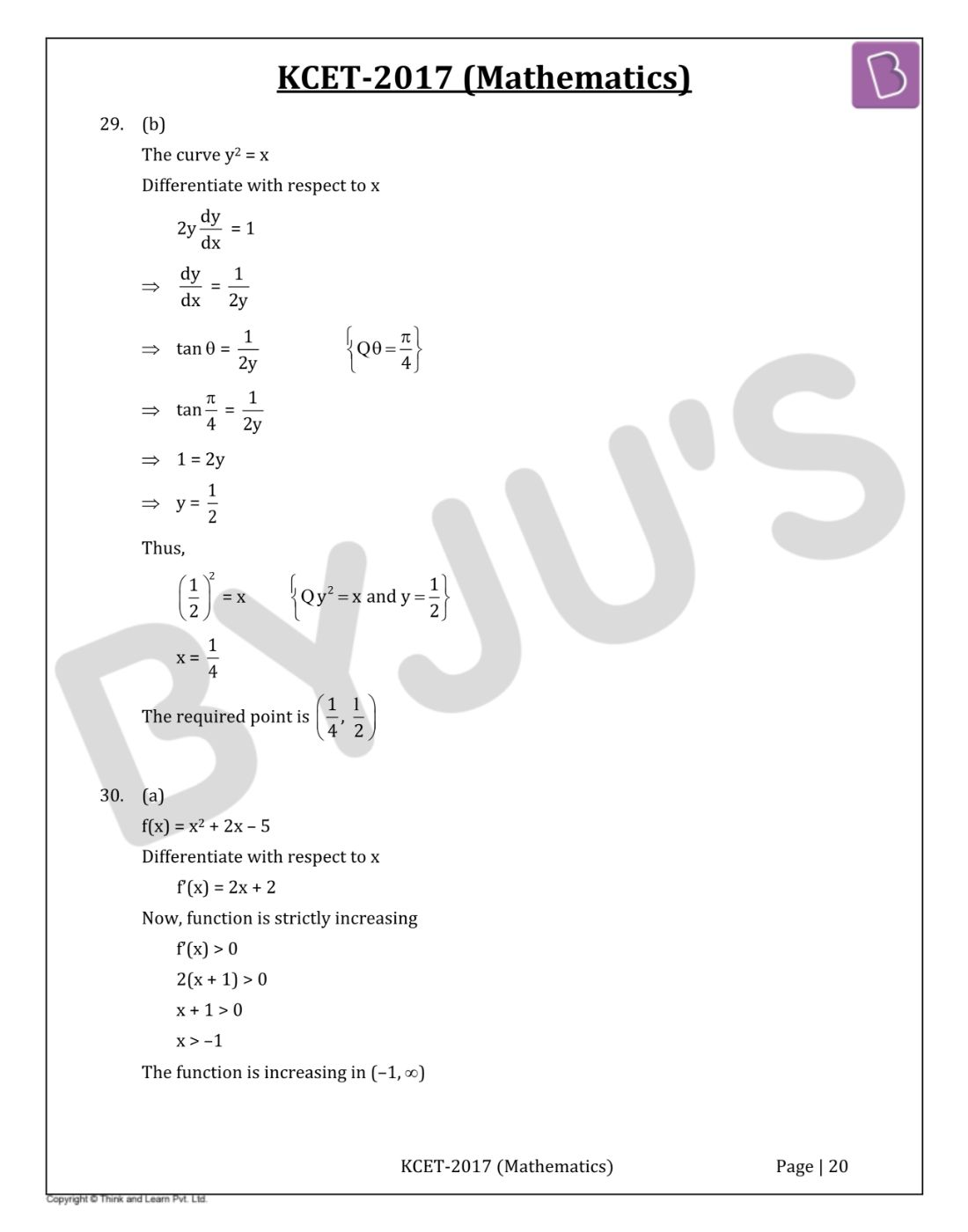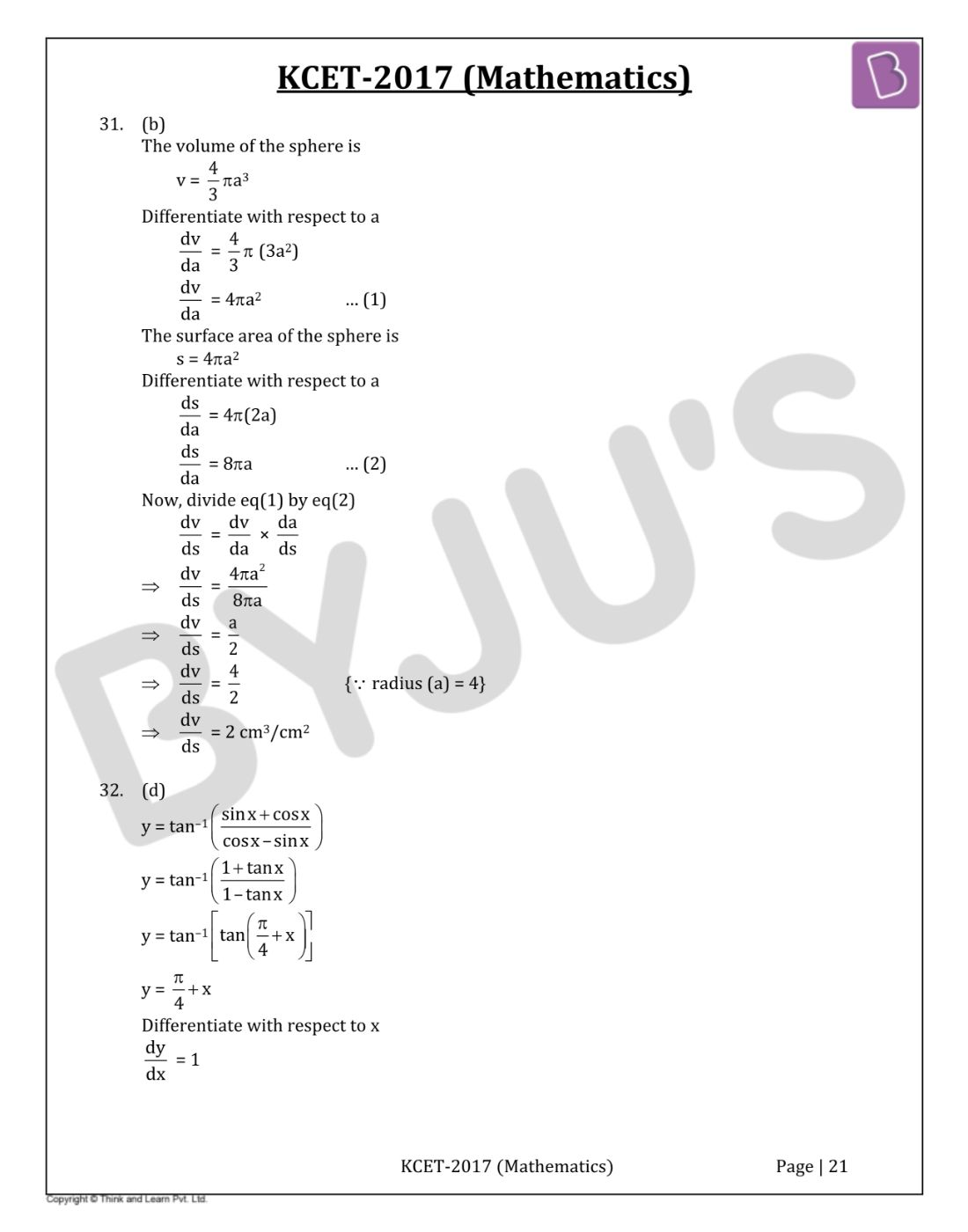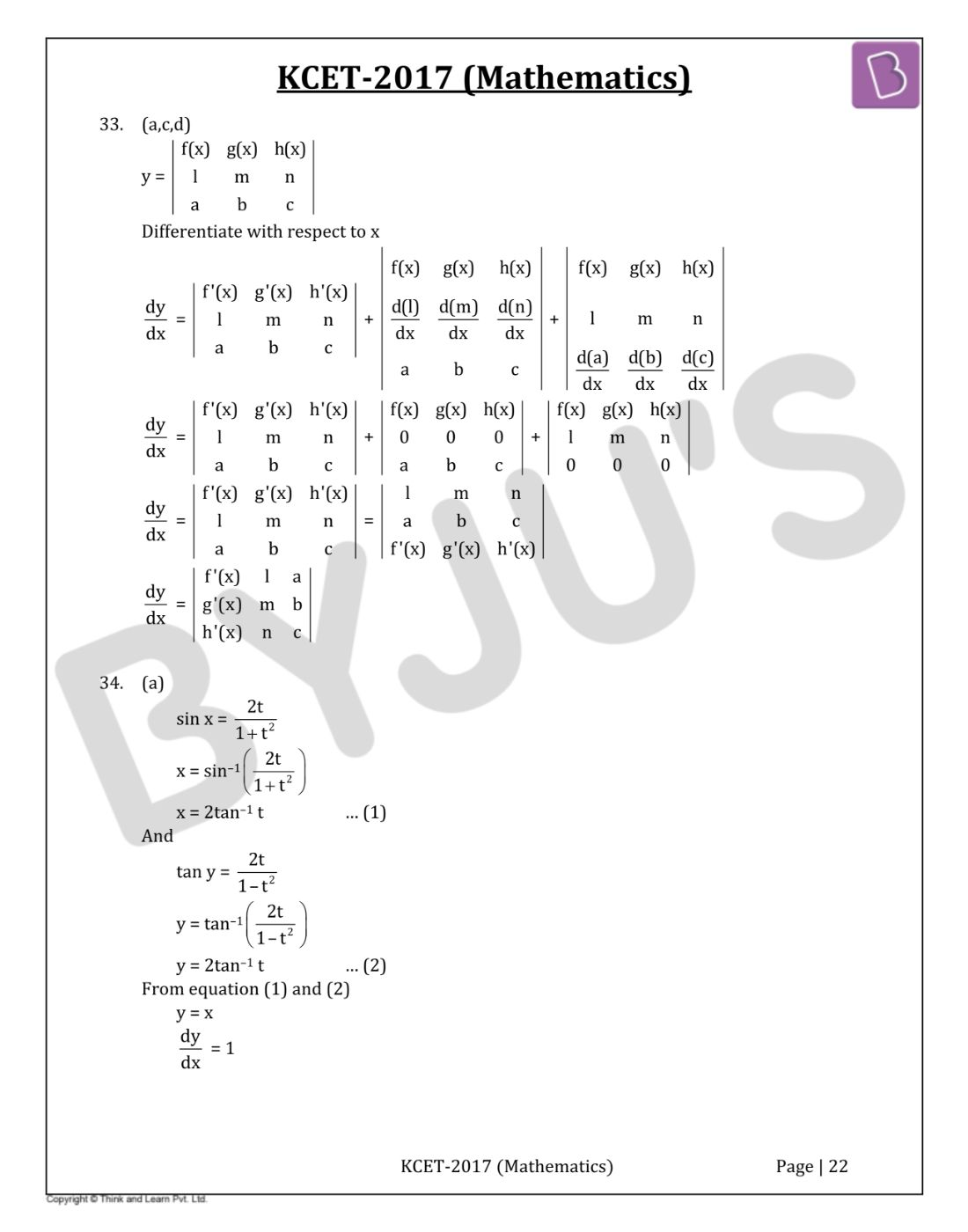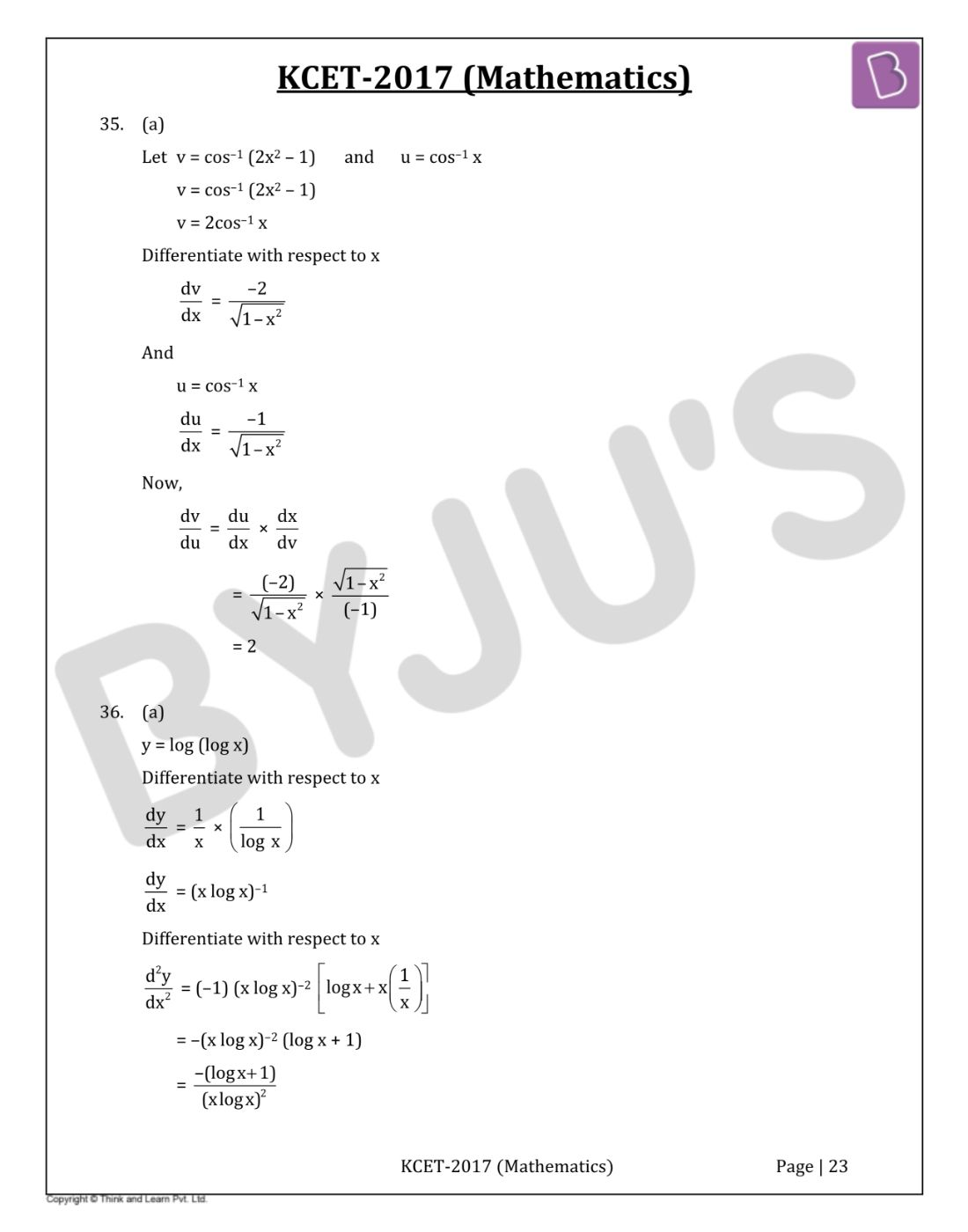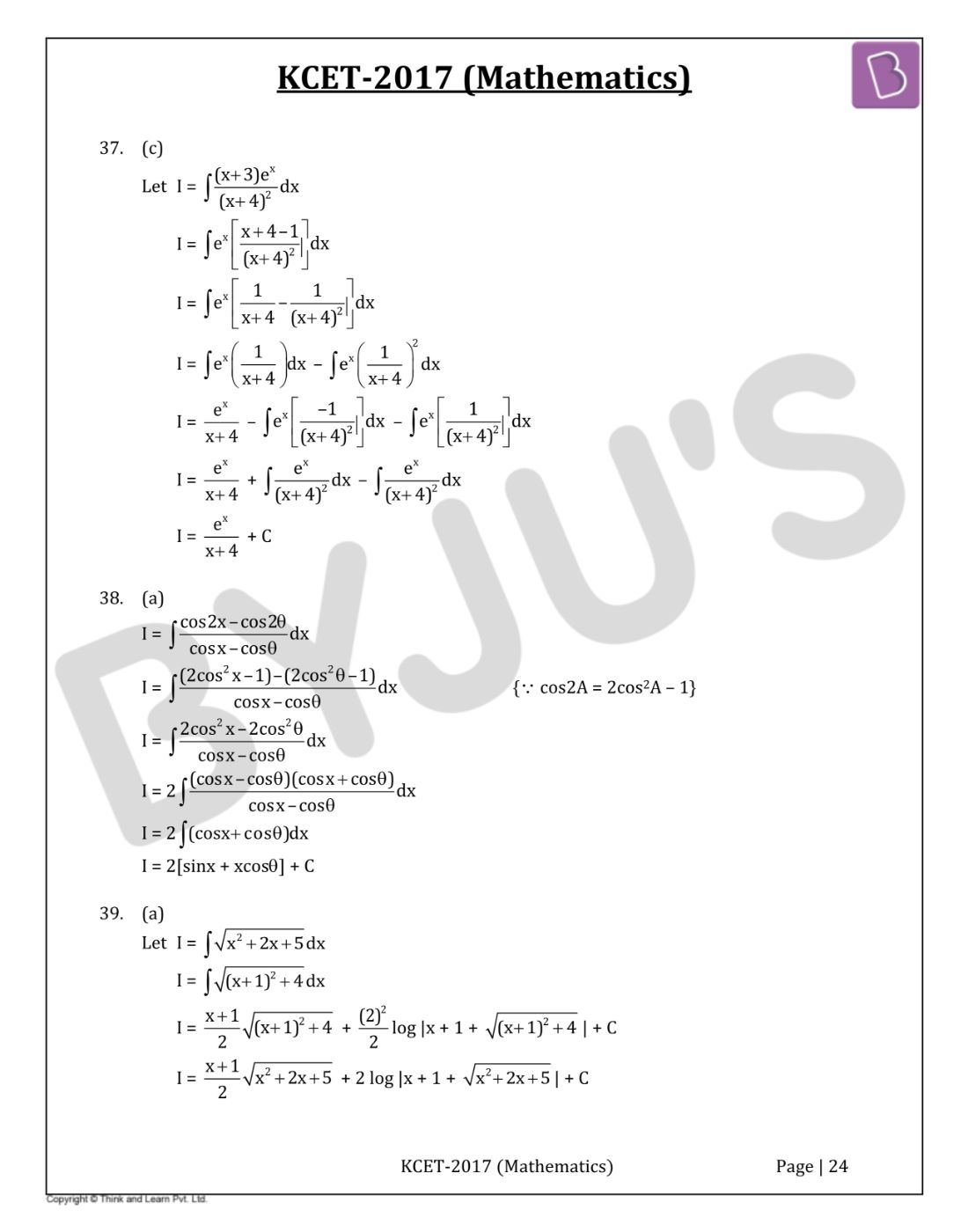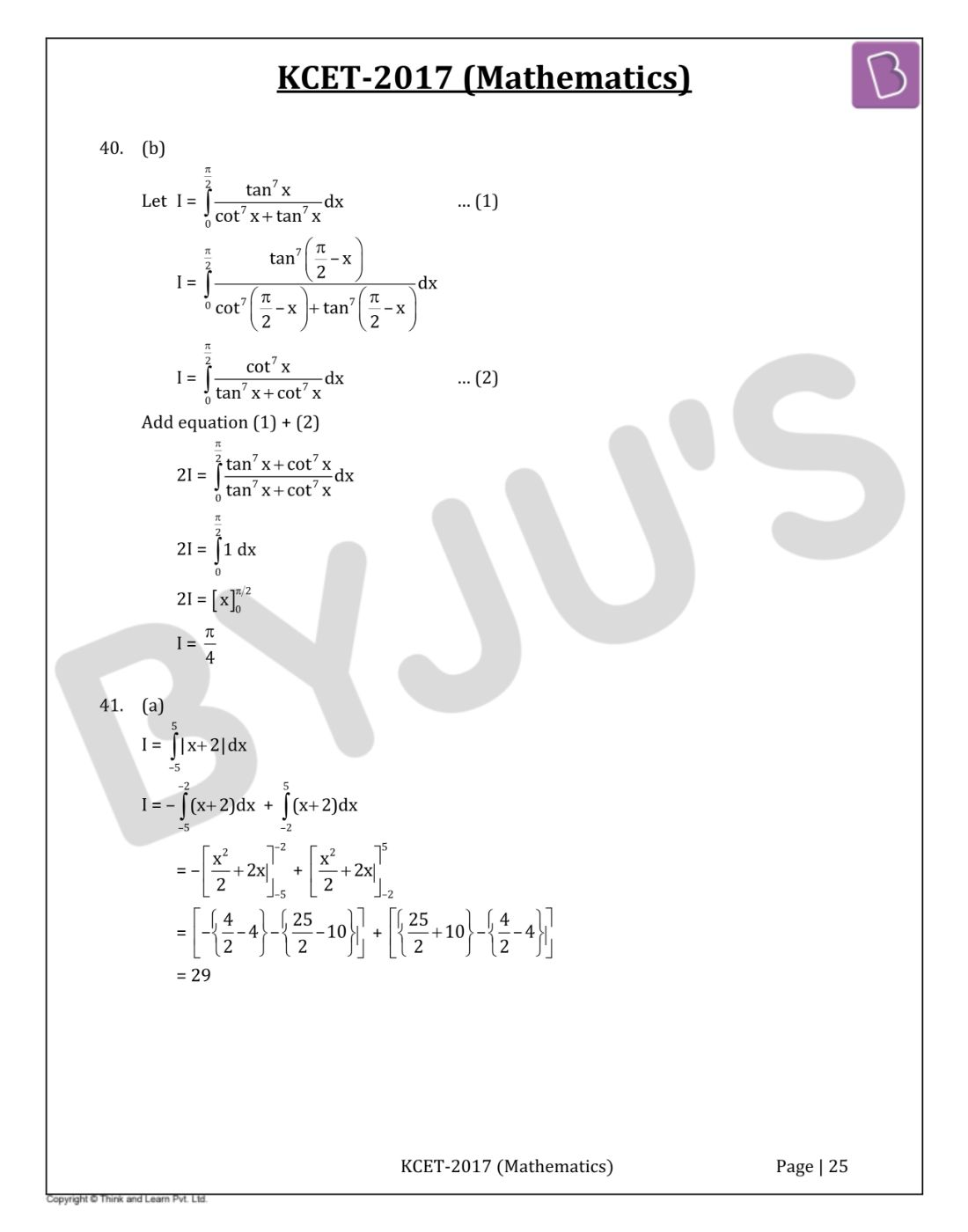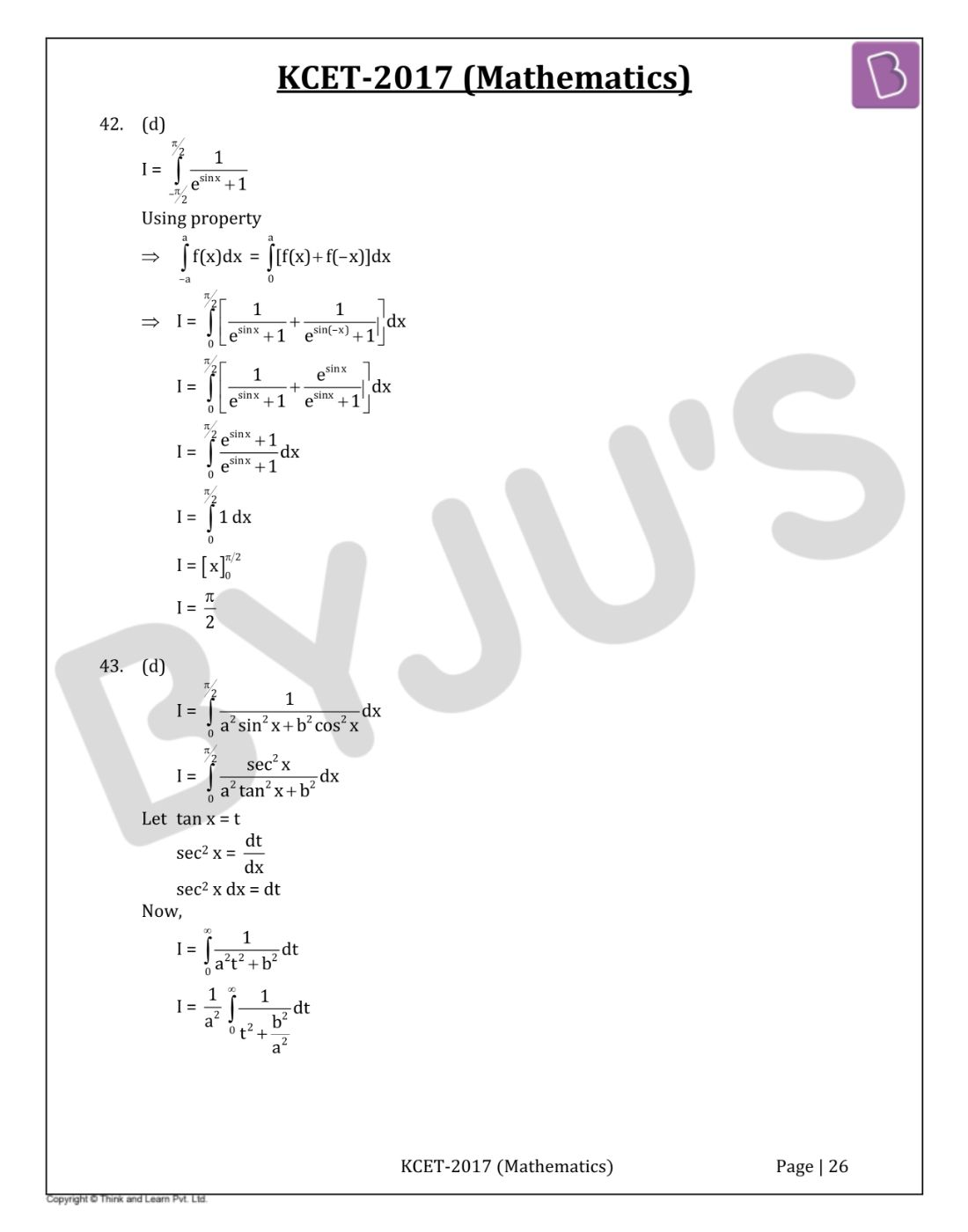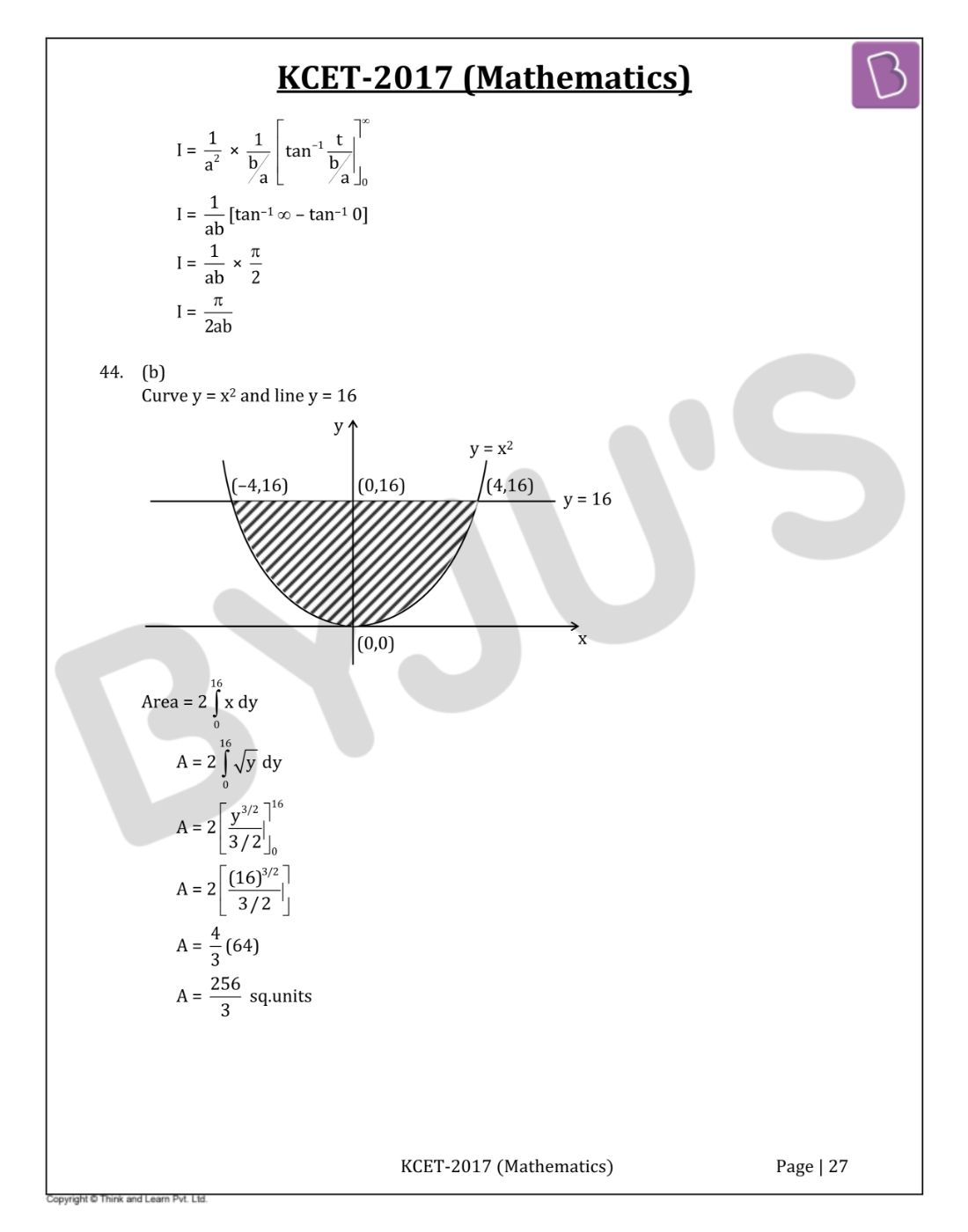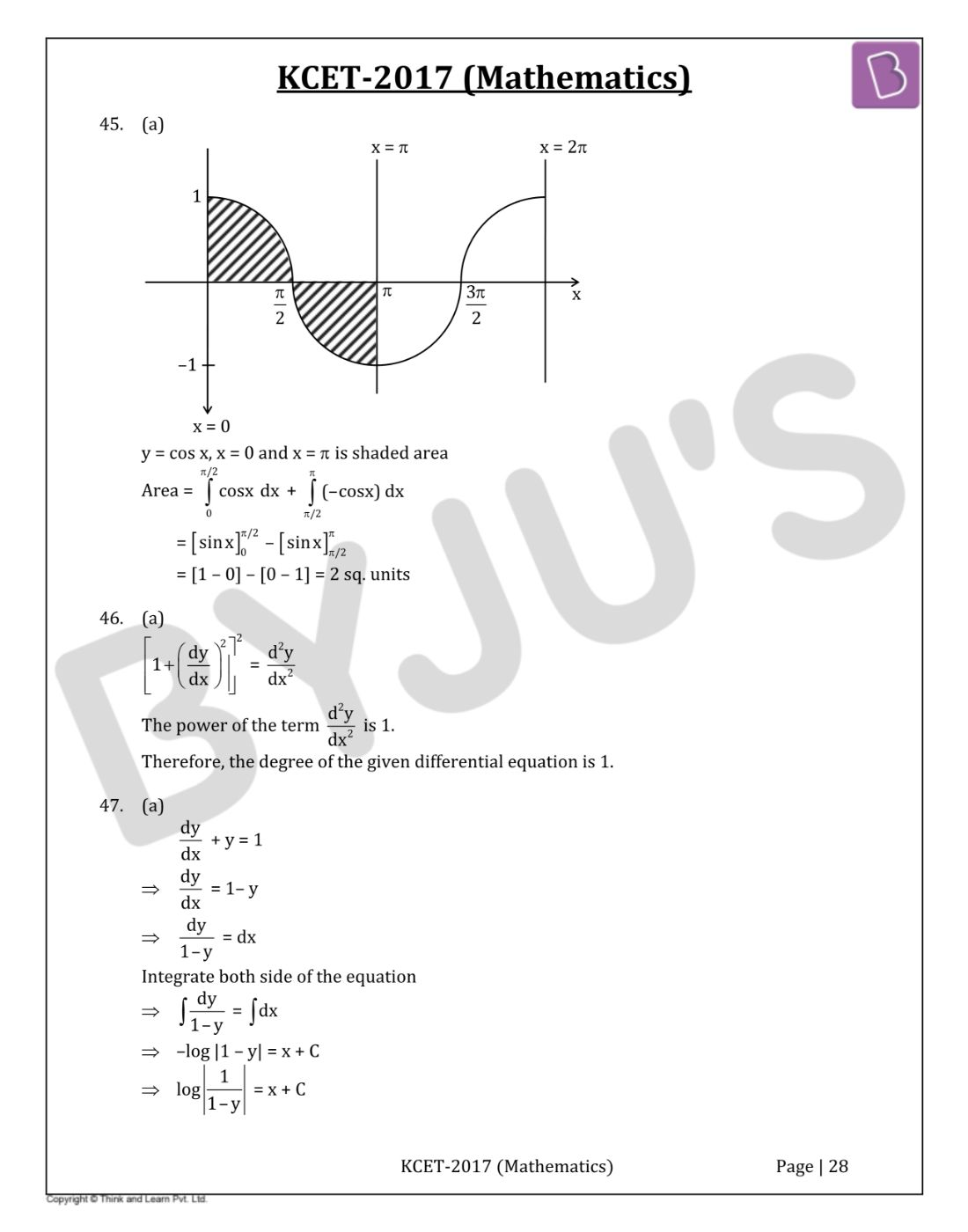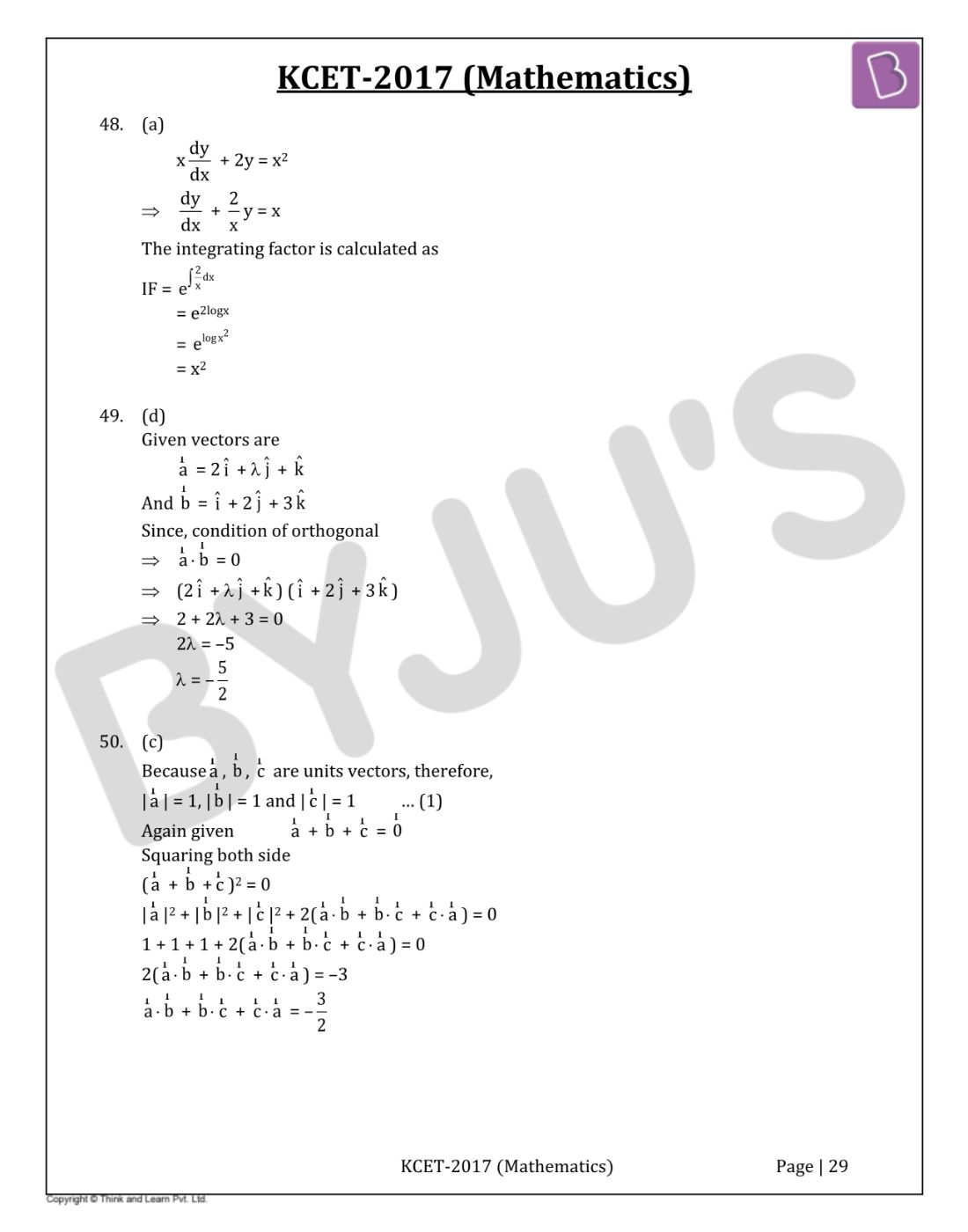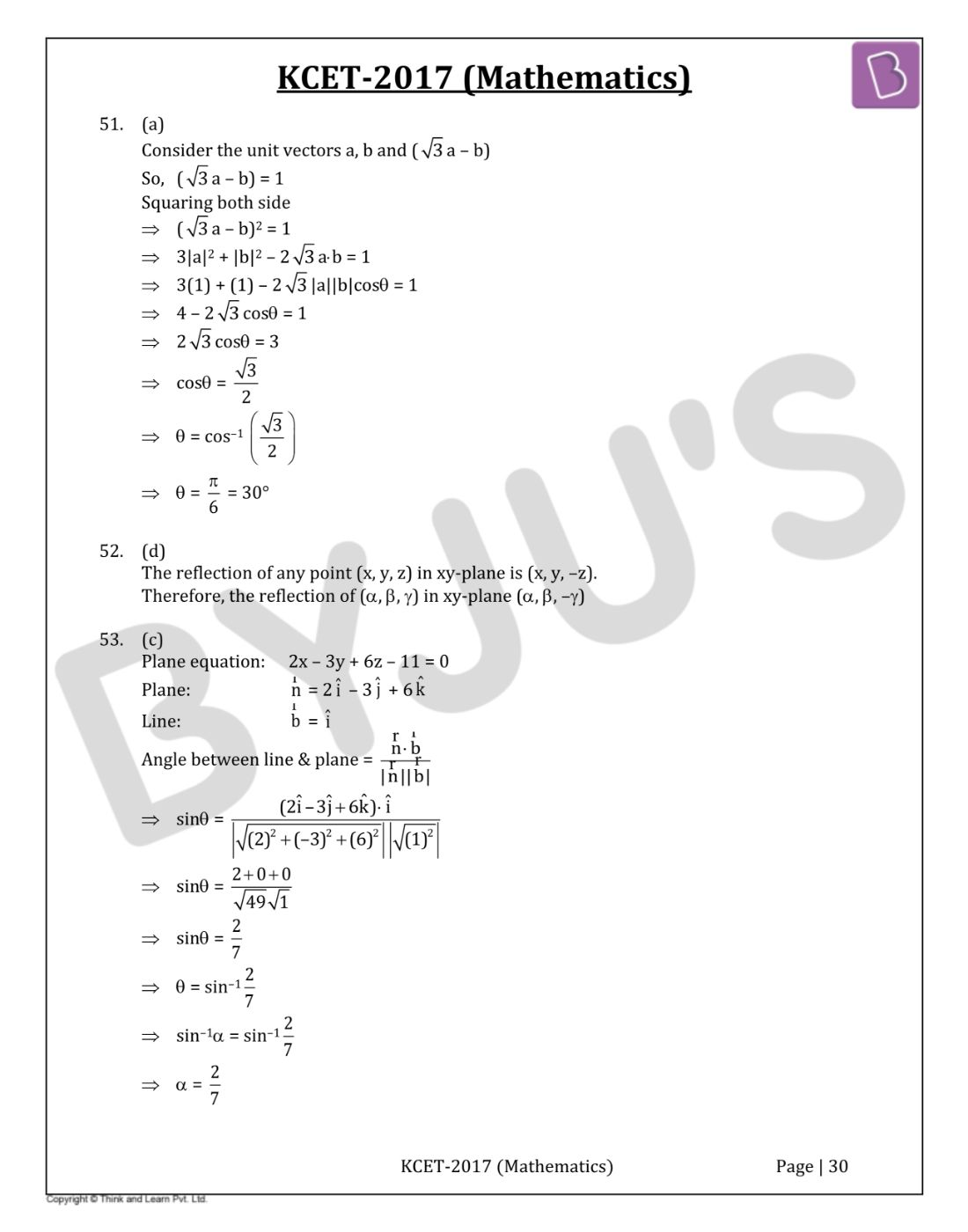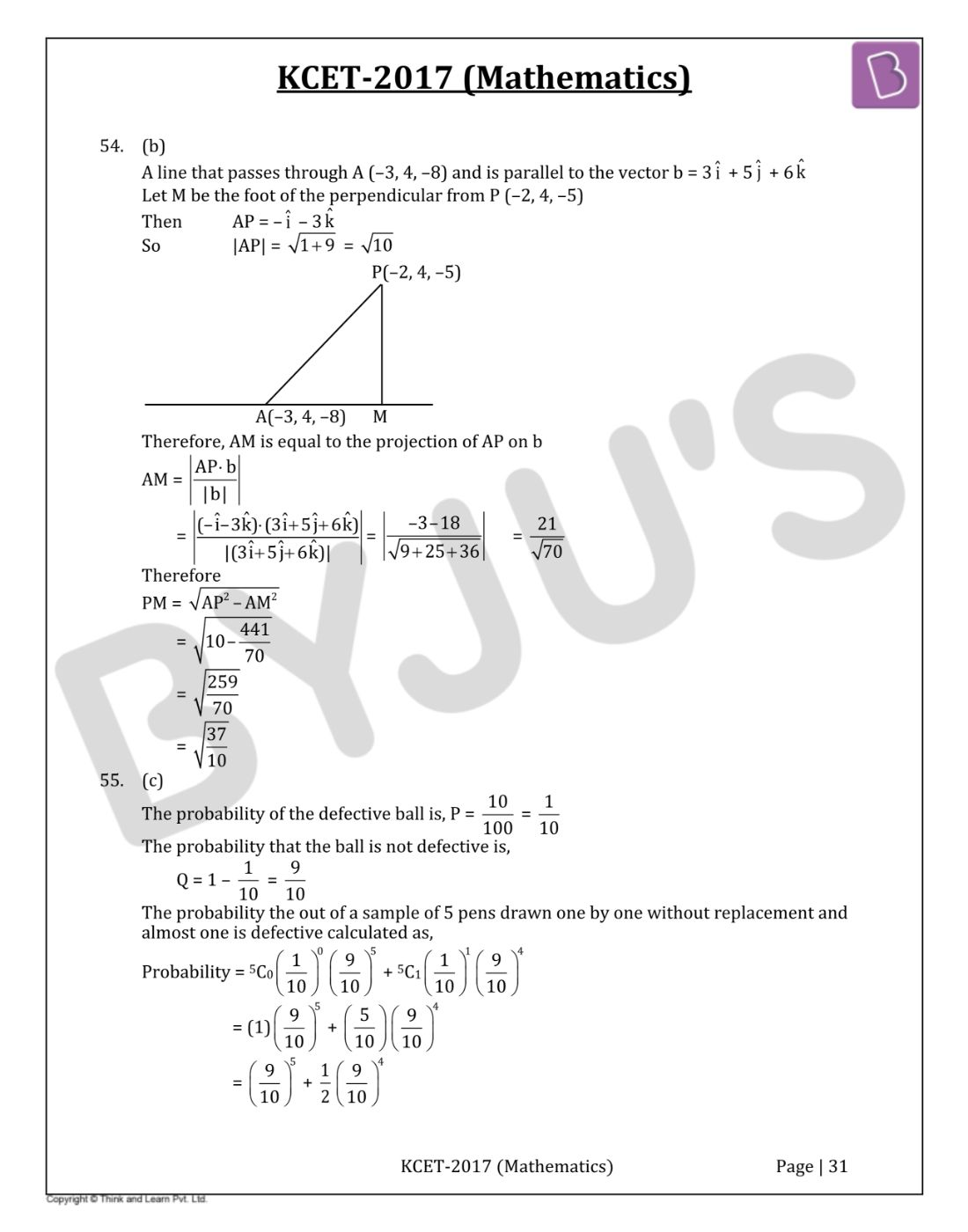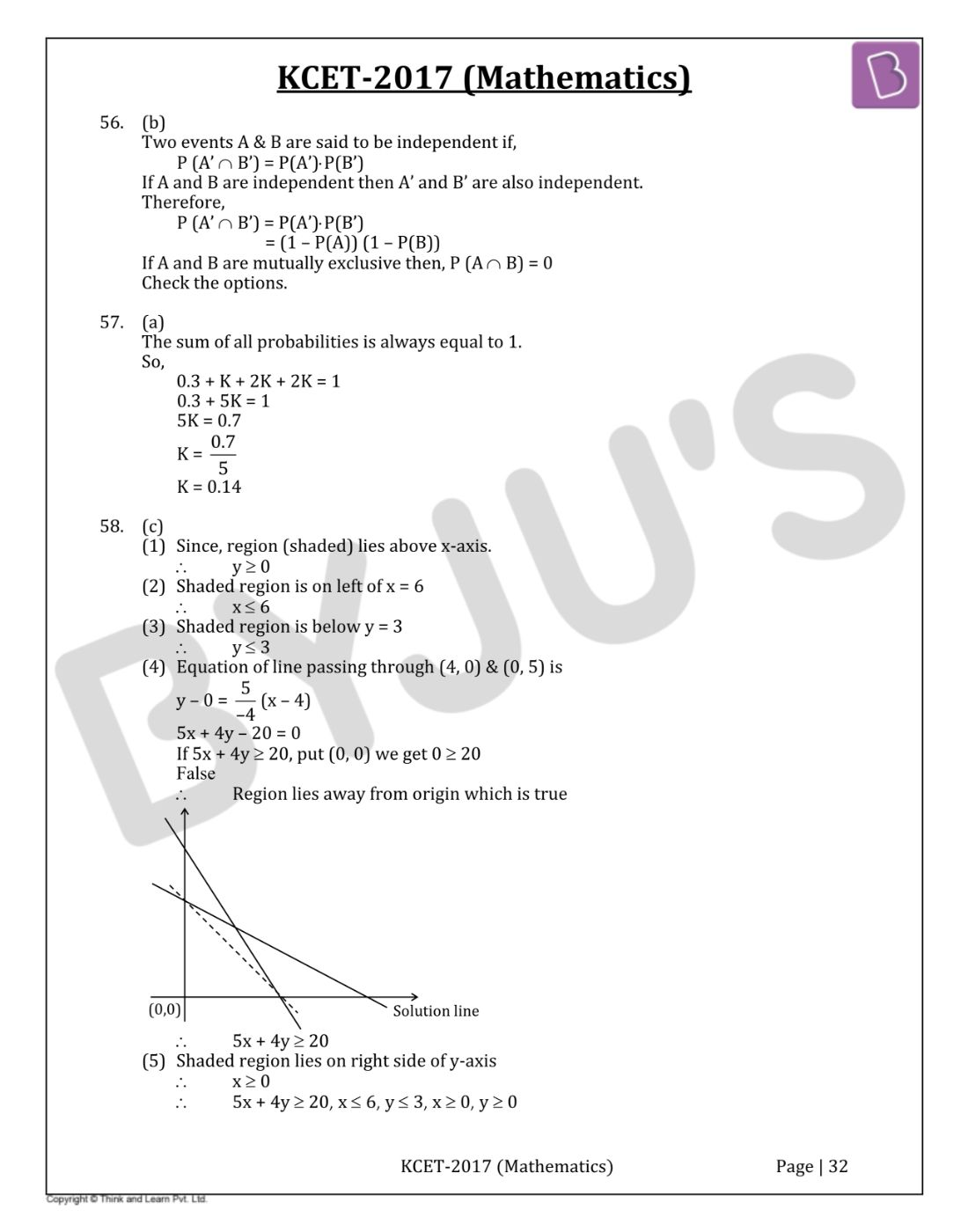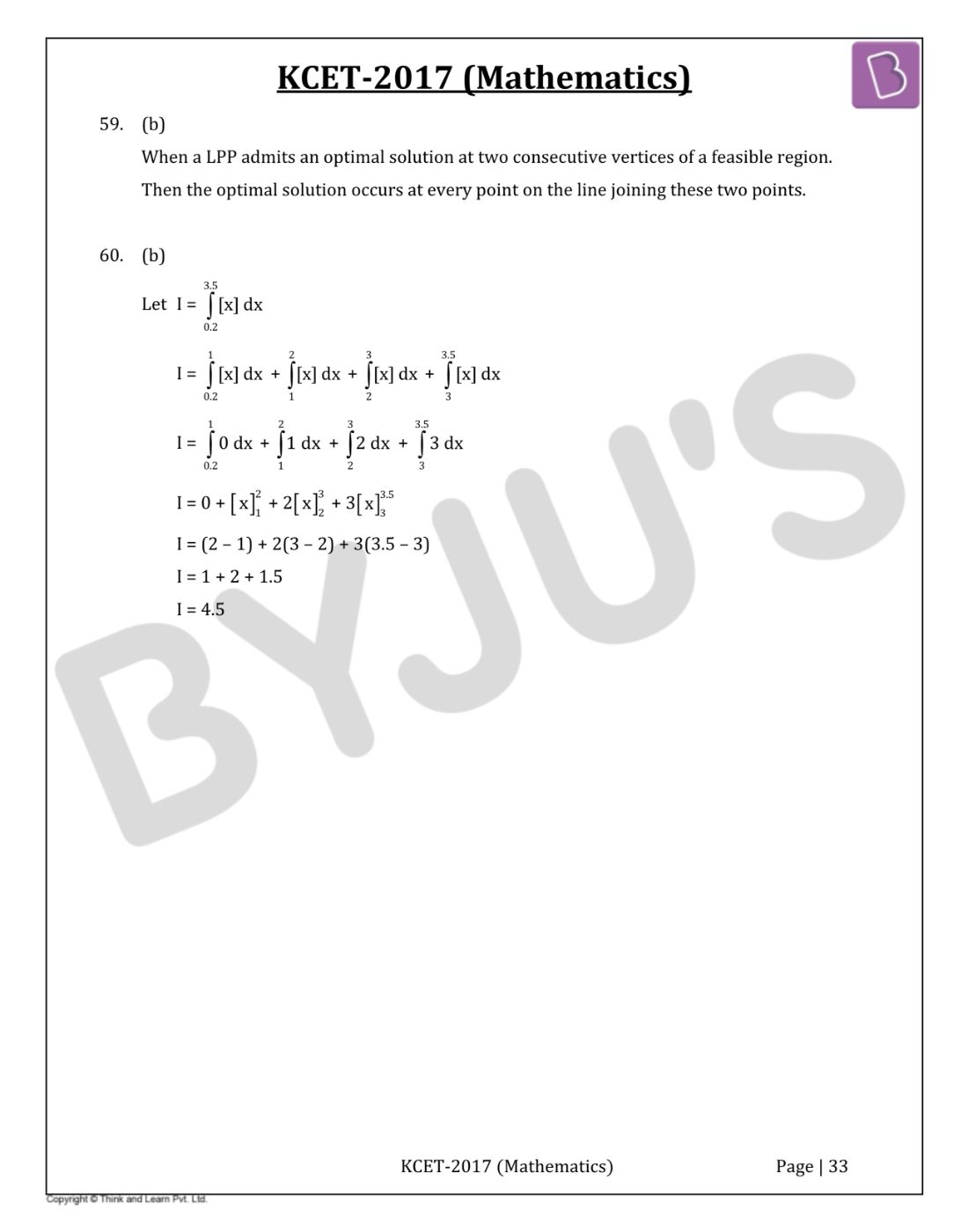# KCET 2017 Maths Paper with Solutions

KCET 2017 Paper with Solutions Maths is available here. It will keep the students in good stead as the exam approaches. It helps the candidates to get an idea on the weightage given to various topics. Candidates can download the previous years’ question papers of KCET to understand the question paper pattern. Practising the previous year papers is the best tool for the preparation of the competitive exam KCET, in terms of solving questions faster and in learning time management. Solving these papers with solutions will help the students in knowing the questions that are frequently asked in the examination.

## KCET 2017 Maths Question Paper with Solutions

KCET 2017 – Maths

Question 1: If A and B are finite sets and A ⊂ B, then

a. n (A ⋃ B) = n (A)
b. n (A ⋂ B) = n (B)
c. n (A ⋃ B) = n (B)
d. n (A ⋂ B) = ϕ

Consider the function A ⊂ B

A ⋂ B = A

N (A ⋂ B) = n (A)

Also,

n (A ⋃ B) = n (A) + n (B) – n (A ⋂ B)

= n (A) + n (B) – n (A)

= n (B)

Question 2: The value of cos2 45o – sin2 15o is –

a. √3 / 2
b. √3 / 4
c. [√3 + 1] / 2 √2
d. [√3 – 1] / 2 √2

cos2 45o – sin2 15o

cos (45o + 15o) cos (45o – 15o) {∵ cos2A – sin2 B = cos (A + B) cos (A – B)}

= cos 60o cos 30o

= (1 / 2) (√3 / 2)

= √3 / 4

Question 3: 3 + 5 + 7 + ……. to n term is –

a. n (n + 2)
b. n (n – 2)
c. n2

d. (n + 1)2

3 + 5 + 7 +……………………………+ n

(a = 3, d = 2)

Sn = (n / 2) [2a + (n – 1)d]

= (n / 2) [2 * 3 + (n – 1) 2]

= (n / 2) (6 + 2n – 2)

= (n / 2) (4 + 2n)

= n (n + 2)

Question 4: If ([1 + i] / [1 – i])m = 1, then the least positive integral value of m is –

a. 2
b. 3
c. 4
d. 1

Consider the equation

([1 + i] / [1 – i])m = 1

([1 + i] / [1 – i]) * ([1 + i] / [1 + i])m = 1

[(1 + i)2 / (1 – i2)]m = 1

[(1 + 2i – 1) / (1 + 1)]m = 1

(2i / 2)m = 1

(i)m = 1

(i)m = 14

m = 4

Question 5: If |x − 2| ≤ 1, then –

a. x ∈ [1, 3]
b. x ∈ (1, 3)
c. x ∈ [- 1, 3)
d. x ∈ (- 1, 3)

|x − 2| ≤ 1

–1 ≤ x – 2 ≤ 1

-1 + 2 ≤ x ≤ 1 + 2

-1 ≤ x ≤ 3

Thus, x ∈ [1, 3]

Question 6: If nC12 = nC8 then n is equal to –

a. 26
b. 12
c. 6
d. 20

It is known that if,

nCx = nCy

Then x + y = n

Now nC12 = nC8

So 12 + 8 = n

n = 20

Question 7: The total number of terms in the expansion of (x + a)47 – (x – a)47 after simplification is –

a. 24
b. 47
c. 48
d. 96

Consider the expansion (x + a)47– (x – a)47

Here, n = 47 which is odd

The total number of the term in the expansion is,

Total term = (n + 1) / 2

= (47 + 1) / 2 = 48 / 2

= 24

Question 8: Equation of line passing through the point (1, 2) and perpendicular to the line y = 3x – 1 is:-

a. x + 3y – 7 = 0
b. x + 3y + 7 = 0
c. x + 3y = 0
d. x – 3y = 0

The equation of line y = 3x – 1

Slope of the line is m = 3

The slope of the line perpendicular to the given line m1 = – 1 / m

m1 = – 1 / 3

The equation of the required line is

(y – y1) = m (x – x1)

(y – 2) = – 1 / 3 (x – 1)

3y – 6 = –x + 1

x + 3y – 7 = 0

Question 9: The eccentricity of the ellipse x2 / 36 + y2 / 16 = 1 is

a. 2√5 / 6
b. 2√5 / 4
c. 2√13 / 6
d. 2√13 / 4

The equation of the ellipse is

x2 / 36 + y2 / 16 = 1

x2 / 62 + y2 / 42 = 1

So, a = 6, b = 4

Eccentricity (e) = √1 – (b2 / a2)

= √1 – (42 / 62) = √1 – (16 / 36) = √(36 – 16) / 36 = √20 / 36 = 2√5 / 6

Question 10: The perpendicular distance of the point P (6, 7, 8) from XY-plane is –

a. 8
b. 7
c. 6
d. 5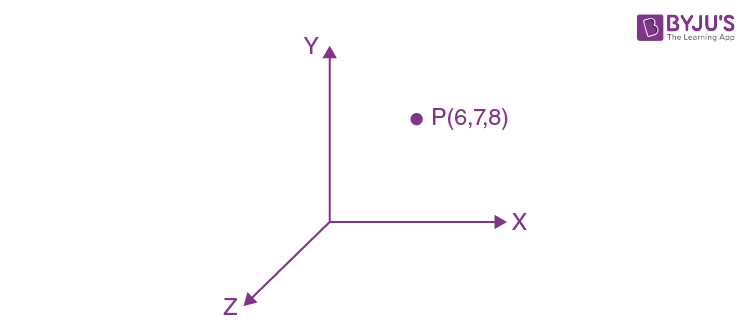The given point is P (6, 7, 8)

Distance of any point from xy-plane = |z|

D = |z|

D = |8|

D = 8

Question 11: The value of lim θ→0 (1 – cos 4θ) / (1 – cos 6θ) is

a. 4 / 9
b. 9 / 4
c. 9 / 3
d. 3 / 4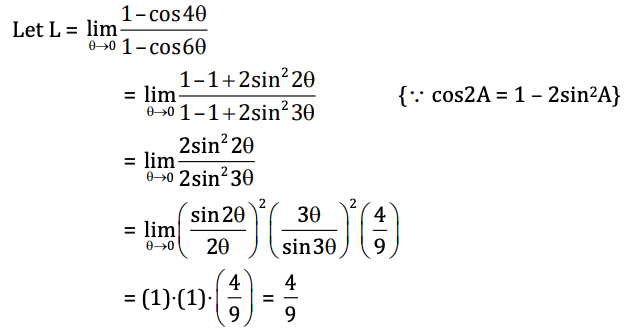Question 12: The contrapositive statement of the statement “If x is a prime number, then x is odd” is –

a. If x is not a prime number, then x is not odd
b. If x is a prime number, then x is not odd
c. If x is not a prime number, then x is odd
d. If x is not odd, then x is not a prime number

The contrapositive statement of the statement “If x is a prime number then x is odd” is “If x is not odd then x is not a prime number.

Question 13: If the coefficient of variation is 60 and the standard deviation is 24, then Arithmetic mean is –

a. 40
b. 7 / 20
c. 20 / 7
d. 1 / 40

The coefficient of variation is CV = 60 and the standard deviation is σ = 24 Coefficient of variation = [σ / mean] × 100

60 = [24 / mean] × 100

Mean = [24 / 60] × 100

Mean = 40

Question 14: The range of the function f (x) = √9 – x2 is

a. (0, 3)
b. [0, 3]
c. (0, 3]
d. [0, 3)

Let y = f (x) = √9 – x2

Squaring both sides,

y2 = 9 – x2

x = √9 – y2

Now,

9 – y2 ≥ 0

(3 – y) (3 + y) ≥ 0

(y – 3) (y + 3) ≤ 0y ∈ [0, 3]

So f (x) ∈ [0, 3]

Question 15: Let f : R → R be defined by f (x) = x4, then –

a. f is one-one and onto
b. f may be one-one and onto
c. f is one-one but not onto
d. f is neither one-one nor onto

Given function f (x) = x4

Let f (x1) = x14

And

f (x2) = x24

So, f (x1) = f (x2)

x14 = x24

x1 = ± x2

So f (x) is not one-one. The co-domain of f (x) is R and the range is f (x) ∈ [0, ∞) Since the co-domain is not equal to the range of the function.

Thus, the function is not onto.

Question 16: The range of sec-1x is –

a. [- π / 2, π / 2]
b. (- π / 2, π / 2)
c. [0, π]
d. [0, π] – {π / 2}

The graph of sec–1 x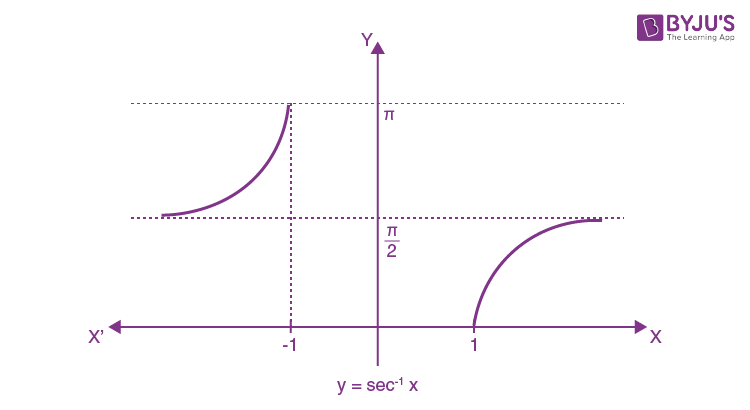The range of the sec–1 x is [0, π] – {π / 2}.

Question 17: If tan-1 x + tan-1 y = 4π / 5, then cot-1 x + cot-1 y is equal to

a. π
b. π / 5
c. 2π / 5
d. 3π / 5

Given function

tan-1 x + tan-1 y = 4π / 5

(π / 2) – cot-1 x + (π / 2) – cot-1 y = 4π / 5 {Q tan-1 x + cot-1 x = π / 2}

cot-1 x + cot-1 y = π – (4π / 5)

cot-1 x + cot-1 y = (π / 5)

Question 18: If f (x) = 8x3, g (x) = x1/3, then fog (x) is

a. 8x
b. 83x
c. (8x)1/3

d. 8x3

Given function f (x) = 8x3

And

g (x) = x1/3

Now, fog(x)

= f [g (x)]

= f [x1/3]

= 8 (x1/3)3

= 8x

Question 19: If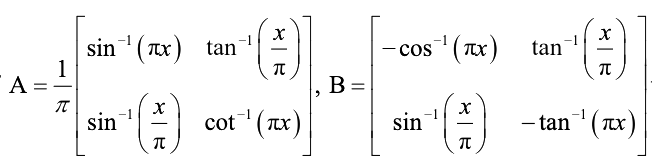then A – B is equal to –

a. I
b. 0
c. 2I
d. (1 / 2)I

Bonus

Question 20: If a matrix A is both symmetric and skew-symmetric, then –

a. A is diagonal matrix
b. A is zero matrix
c. A is scalar matrix
d. A is a square matrix

Let A be a matrix that is both symmetric and skew-symmetric.

Therefore,

A’ = A

And A’ = –A

This case is only possible when A is a zero matrix.

Question 21: If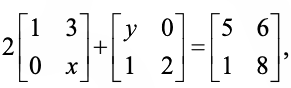then the value of x and y are –

a. x = 3, y = 3
b. x = -3, y = 3
c. x = 3, y = -3
d. x -3, y = -3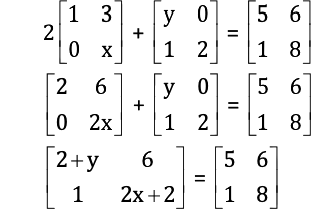So

2 + y = 5

y = 3

2x + 2 = 8

2x = 6

x = 3

Question 22: Binary operation * on R – {-1} defined by a * b = a / (b + 1) is –

a. * is associative and commutative
b. * is associative but not commutative
c. * is neither associative nor commutative
d. * is commutative but not associative

Given that a * b = a / (b + 1) — (1)

So,

b * a = b / (a +1) —- (2)

Thus a * b ≠ b * a

Hence, the operation is not commutative.

Now,

a * (b * c) = a * (b / [c + 1])

= a / {[(b / (c + 1)] + 1}

= a (c + 1) / [b + c + 1]

and

a * (b * c) = (a / [b + 1]) * c

= a / {[b + 1] / [c + 1]}

= a / (b + 1) (c + 1)

So a * (b * c) = (a * b) * c

Hence, the operation is not associative.

Question 23: If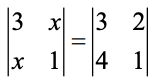, then x is equal to –

a. 2
b. 4
c. 8
d. 2√2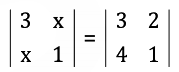3 – x2 = 3 – 8

–x2 = –8

x2 = 8

x = ± 2√2

Question 24: If A is a square matrix of order 3 × 3, then |KA| is equal to –

a. K |A|
b. K2 |A|
c. K3 |A|
d. 3K |A|

For a n × n order matrix of degree 2.

|KA| = Kn |A|

Here n is the order of the matrix.

Since the matrix of the 3 × 3,

|KA| = K3 |A|

Question 25: The area of a triangle with vertices (K, 0), (4, 0), (0, 2) is 4 square units, then the value of K is –

a. 0 or 8
b. 0 or -8
c. 0
d. 8

The vertices of the triangle are (K, 0), (4, 0), (0, 2).

The area of the triangle is 4 sq. units.

Δ = (1 / 2)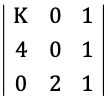4 = (1 / 2) |[K (0 – 2) – 0(4 – 0) + 1(8 – 0)]|

4 = (1 / 2) |–2K + 8]| ⇒ 8 = |–2K + 8|

–2K + 8 = ± 8

Now, –2K + 8 = 8

–2K = 0

K = 0

And

–2K + 8 = –8

–2K = –16;

K = 8

Question 26: Let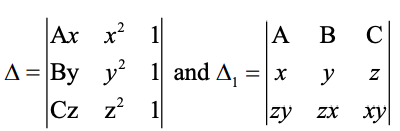, then

a. Δ1 = − Δ
b. Δ1 = Δ
c. Δ1 ≠ Δ
d. Δ1 = 2Δ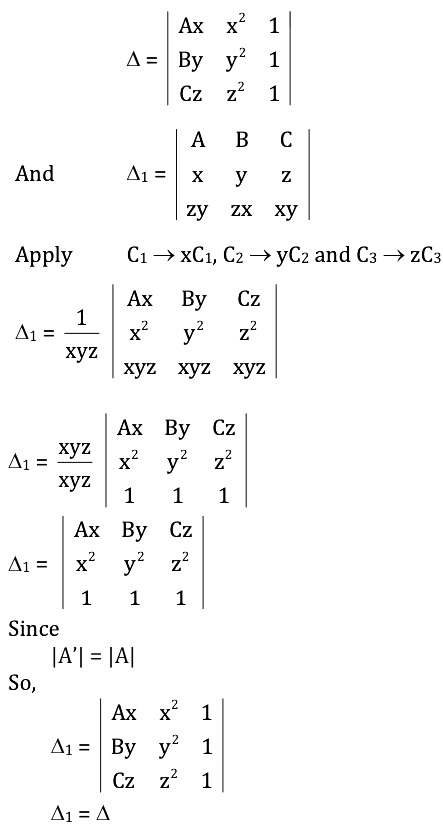Question 27: If f (x) =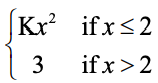is continuous at x = 2, then the value of K is –

a. 3
b. 4
c. 3 / 4
d. 4 / 3

f (x) =The function is continuous at x = 2. So,

lim x→2 Kx2 = lim x→2 3

K(2)2 = 3

K = 3 / 4

Question 28: The value of C in Mean value theorem for the function f (x) = x2 in [2, 4] is –

a. 3
b. 2
c. 4
d. 7 / 2

The mean value theorem is given as

f ‘ (c) = [f (b) – f (a)] / [b – a]

Now, f (x) = x2; f’(x) = 2x

Therefore

f ‘ (c) = [f (4) – f (2)] / [4 – 2]

2c = [16 – 4] / 2

c = 12 / 4

= 3

Question 29: The point on the curve y2 = x where the tangent makes an angle of π / 4 with the x-axis is –

a. 1 / 2, 1 / 4
b. 1 / 4, 1 / 2
c. (4, 2)
d. (1, 1)

The curve y2 = x

Differentiate with respect to x

2y (dy / dx) = 1

(dy / dx) = 1 / 2y

tan θ = 1 / 2y {Qθ = π / 4}

tan [π / 4] = 1 / 2y

1 = 2y

y = 1 / 2

Thus,

(1 / 2)2 = x {Qy2 = x and y = 1 / 2}

x = 1 / 4

The required point is (1 / 4, 1 / 2).

Question 30: The function f (x) = x2 + 2x – 5 is strictly increasing in the interval –

a. (− 1, ∞)
b. (− ∞, − 1)
c. [– 1, ∞)
d. (− 1, ∞)

f (x) = x2 + 2x – 5

Differentiate with respect to x

f’(x) = 2x + 2

Now, function is strictly increasing

f’(x) > 0

2(x + 1) > 0

x + 1 > 0

x > –1

The function is increasing in (–1, ∞).

Question 31: The rate of change of a sphere with respect to its surface area when the radius is 4 cm is –

a. 4 [cm3 / cm2]
b. 2 [cm3 / cm2]
c. 6 [cm3 / cm2]
d. 8 [cm3 / cm2]

The volume of the sphere is v = (4 / 3) πa3

Differentiate with respect to a,

dv / da = (4 / 3) π (3a2)

dv / da = 4πa2 … (1)

The surface area of the sphere is s = 4πa2

Differentiate with respect to a

ds / da = 4π(2a)

ds / da = 8πa … (2)

Now, divide equation (1) by equation (2)

dv / ds = [dv / da] × [da / ds]

dv / ds = 4πa2 / 8πa

dv / ds = a / 2

dv / ds = 4 / 2 { radius (a) = 4}

dv / ds = 2 [cm3 / cm2]

Question 32: If y = tan-1 [(sinx + cosx) / cosx – sinx)], then (dy / dx) is equal to –

a. 1 / 2
b. π / 4
c. 0
d. 1

y = tan-1 [(sinx + cosx) / cosx – sinx)]

y = tan-1 [(1 + tanx) / (1 – yanx)]

y = tan-1 [(tan (π / 4) + x)]

y = (π / 4) + x

Differentiate with respect to x,

dy / dx = 1

Question 33: If y =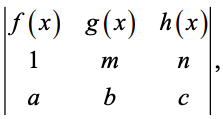then dy / dx is equal to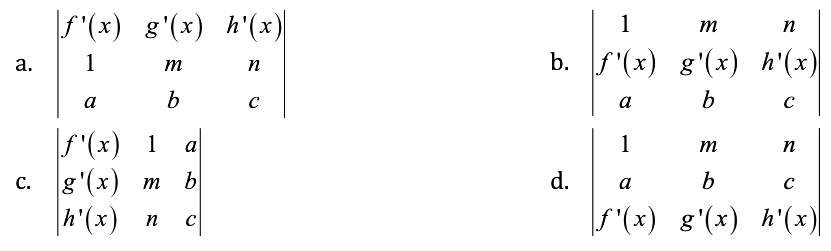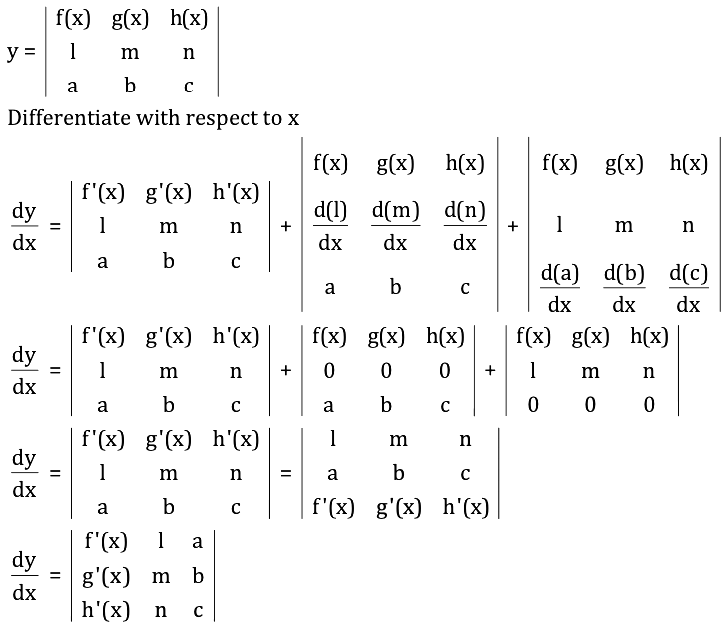Question 34: If sinx = 2t / (1 + t2), tany = 2t / (1 – t2), then dy / dx is equal to

a. 1
b. 0
c. -1
d. 2

sinx = 2t / (1 + t2)

x = sin-1 [2t / (1 + t2)]

x = 2 tan-1 t —- (1)

tany = 2t / (1 – t2)

y = tan-1 [2t / (1 – t2)]

y = 2 tan–1 t —- (2)

From equation (1) and (2)

y = x

(dy / dx) = 1

Question 35: The derivative of cos-1 (2x2 – 1) w.r.t 1 cos-1 x is –

a. 2
b. -1 / (2 √1 – x2))
c. 2 / x
d. 1 – x2

Let v = cos–1 (2x2 – 1) and u = cos–1 x

v = cos–1 (2x2 – 1)

v = 2 cos–1 x

Differentiate with respect to x

dv / dx = -2 / √1 – x2

And

u = cos–1 x

du / dx = -1 / √1 – x2

Now,

dv / du = [du / dx] × [dx / dv]

= [-2 / √1 – x2] × [√1 – x2 / -1]

= 2

Question 36: If y = log (log x) then d2y / dx2 is equal to –

a. – [1 + logx] / (x logx)2

b. – [1 + logx] / x2 logx
c. [1 + logx] / (x logx)2

d. [1 + logx] / x2 logx

y = log (log x)

Differentiate with respect to x

dy / dx = (1 / x) × (1 / logx)

= (x logx)-1

Differentiate with respect to x

d2y / dx2 = (-1) (x logx)-2 [logx + x (1 / x)]

= – (x log x)–2 (log x + 1)

= – [1 + logx] / (x logx)2

Question 37: ∫[(x + 3)ex / (x + 4)2] dx is equal to

a. (1 / [x + 4]2) + c
b. (ex / [x + 4]2) + c
c. (ex / [x + 4]) + c
d. (ex / [x + 3]) + c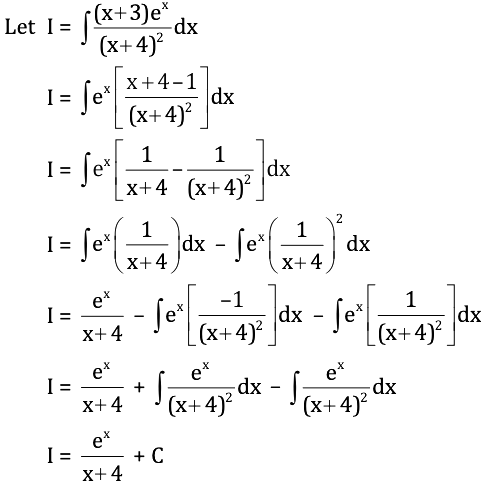Question 38: ∫[cos 2x – cos 2θ] / [cosx – cos θ] is equal to

a. 2 (sinx + x cos θ) + c
b. 2 (sinx – x cos θ) + c
c. 2 (sinx + 2x cos θ) + c
d. 2 (sinx – 2x cos θ) + c

$$\begin{array}{l}\\I=\int \frac{cos2x-cos2\Theta}{cosx-cos\Theta}dx\\ I=\int \frac{2cos^{2}x-1-(2cos^{2}\Theta -1)}{cosx-cos\Theta}dx\\ I=\int \frac{2cos^{2}x-2cos^{2}\Theta}{cosx-cos\Theta}dx\\ I=2 \int \frac{(cosx-cos\Theta)(cosx+cos\Theta)}{cosx-cos\Theta}dx\\ I=2\int (cosx+cos\Theta) dx\\ I=\int 2 [sinx+xcos\theta]+c\end{array}$$

Question 39: ∫√x2 + 2x + 5 dx is equal to

a.

$$\begin{array}{l}\frac{1}{2}(x+1)\sqrt{x^{2}+2x+5}+2log|x+1+\sqrt{x^{2}+2x+5}|+c\\ \end{array}$$

b.
$$\begin{array}{l}(x+1)\sqrt{x^{2}+2x+5}+2log|x+1+\sqrt{x^{2}+2x+5}|+c\\ \end{array}$$

c.
$$\begin{array}{l}(x+1)\sqrt{x^{2}+2x+5}-2log|x+1+\sqrt{x^{2}+2x+5}|+c\\ \end{array}$$

d.
$$\begin{array}{l}(x+1)\sqrt{x^{2}+2x+5}+(1/2)log|x+1+\sqrt{x^{2}+2x+5}|+c\end{array}$$

Let I = ∫√x2 + 2x + 5 dx

I = ∫√(x + 1)2 + 4 dx

I = [(x + 1) / 2] √(x + 1)2 + 4 + [(22) / 2] log |x + 1 + √(x + 1)2 + 4| + c

$$\begin{array}{l}\\a.\frac{1}{2}(x+1)\sqrt{x^{2}+2x+5}+2log|x+1+\sqrt{x^{2}+2x+5}|+c\\\end{array}$$

Question 40: 0π/2 [tan7 x / (cot7 x + tan7 x)] dx is equal to –

a. π / 2
b. π / 4
c. π / 6
d. π / 3

$$\begin{array}{l}\\I=\int_{0}^{\frac{\pi}{2}}\frac{tan^{7}x}{cot^{7}x+tan^{7}x}dx —- (1)\\ I=\int_{0}^{\frac{\pi}{2}}\frac{tan^{7}(\frac{\pi}{2}-x)}{cot^{7}(\frac{\pi}{2}-x)+tan^{7}(\frac{\pi}{2}-x)}dx\\ I=\int_{0}^{\frac{\pi}{2}}\frac{cot^{7}x}{tan^{7}x+cot^{7}x}dx —- (2)\\ (1)+(2)\\ 2I=\int_{0}^{\frac{\pi}{2}}\frac{tan^{7}x+cot^{7}x}{tan^{7}x+cot^{7}x}dx\\ 2I=\int_{0}^{\frac{\pi}{2}}1 dx\\ 2I=[x]_{0}^{\frac{\pi}{2}}\\\\ I=\frac{\pi}{4}\\\end{array}$$

Question 41: -55 |x + 2| dx is equal to:

a. 29
b. 28
c. 27
d. 30

$$\begin{array}{l}\\I=\int_{-5}^{5}|x+2|dx\\ I=-\int_{-5}^{-2}(x+2)dx+\int_{-2}^{5}(x+2)dx\\ =-[\frac{x^{2}}{2}+2x]_{-5}^{-2}+[\frac{x^{2}}{2}+2x]_{-2}^{5}\\ =-[({\frac{4}{2}-4})-({\frac{25}{2}}-10)]+[({\frac{25}{2}+10})-({\frac{4}{2}}-4)]\\ =29\end{array}$$

Question 42: -π/2π/2 [dx / (esinx + 1)] is equal to

a. 0
b. 1
c. – (π / 2)
d. (π / 2)

$$\begin{array}{l}\\I=\int_{\frac{-\pi}{2}}^{\frac{\pi}{2}}\frac{1}{e^{sinx}+1}dx\\ \int_{-a}^{a}f(x)dx=\int_{0}^{a}[f(x)+ f(-x)]dx\\ I=\int_{0}^{\frac{\pi}{2}}[\frac{1}{{e^{sinx}+1}}+\frac{1}{{e^{sin(-x)}+1}}]dx\\ I=\int_{0}^{\frac{\pi}{2}}[\frac{1}{{e^{sinx}+1}}+\frac{e^{sinx}}{{e^{sinx}+1}}]dx\\ I=\int_{0}^{\frac{\pi}{2}}\frac{e^{sinx}+1}{e^{sinx}+1}dx\\ I=\int_{0}^{\frac{\pi}{2}}1dx\\ I=[x]_{0}^{\frac{\pi}{2}}\\\\ I={\frac{\pi}{2}}\end{array}$$

Question 43:

$$\begin{array}{l}\\I=\int_{0}^{\frac{\pi}{2}}\frac{1}{a^{2}sin^{2}x+b^{2}cos^{2}x}dx\end{array}$$
is equal to

a. πa / 4b
b. πa / 2b
c. πb / 4a
d. π / 2ab

$$\begin{array}{l}\\I=\int_{0}^{\frac{\pi}{2}}\frac{1}{a^{2}sin^{2}x+b^{2}cos^{2}x}dx\\ I=\int_{0}^{\frac{\pi}{2}}\frac{sec^{2}x}{a^{2}tan^{2}x+b^{2}}dx\\ tanx=t\\ sec^{2}xdx=dt\\ I=\int_{0}^{\infty}\frac{1}{a^{2}t^{2}+b^{2}}dt\\ I=\frac{1}{a^{2}}\int_{0}^{\infty}\frac{1}{t^{2}+\frac{b^{2}}{a^{2}}}dt\\ =\frac{1}{a^{2}}\frac{1}{\frac{b}{a}}[tan^{-1}\frac{t}{\frac{b}{a}}]_{0}^{\infty}\\ =\frac{1}{ab}[tan^{-1}\infty-tan^{-1}0]\\=\frac{1}{ab}\times \frac{\pi}{2}\\ =\frac{\pi}{2ab}\end{array}$$

Question 44: The area of the region bounded by the curve y = x2 and the line y = 16 is –

a. [32 / 3] sq. units
b. [256 / 3] sq. units
c. [64 / 3] sq. units
d. [128 / 3] sq. units

Curve y = x2 and line y = 16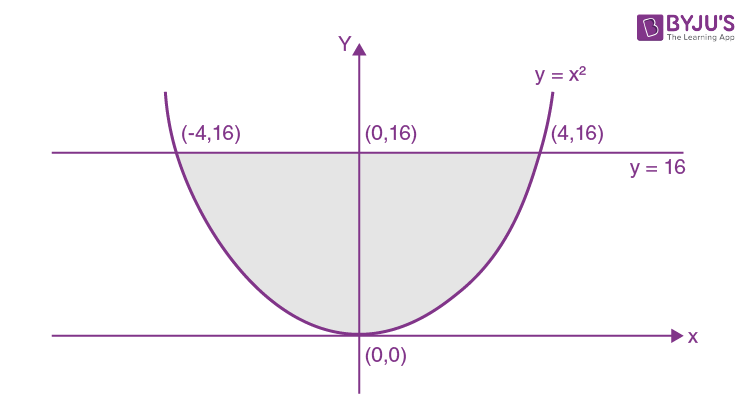Area = 2 ∫016 x dy

A = 2 ∫016 √y dy

= 2 [y3/2 / (3 / 2)]016

= 2 [163/2 / (3 / 2)]

= (4 / 3) * 64

= [256 / 3] sq.units

Question 45: Area of the region bounded by the curve y = cos x , x = 0 and x = π is –

a. 2 sq. units
b. 4 sq. units
c. 3 sq. units
d. 1 sq. unit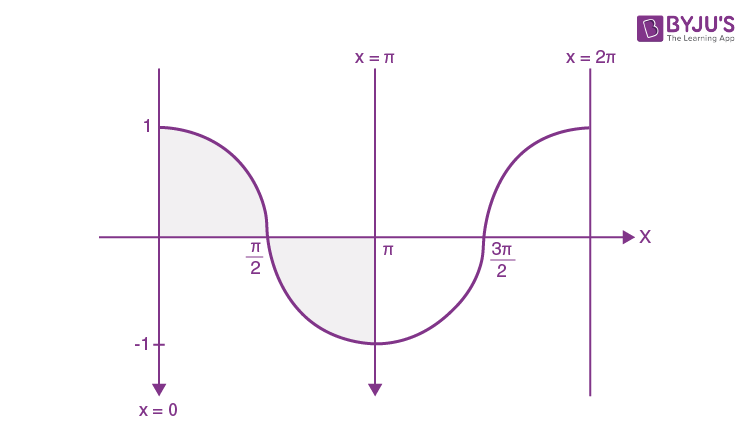y = cos x, x = 0 and x = π is shaded area

A = ∫0π/2 cosx dx + ∫ππ/2 (–cosx) dx

= [sinx]0π/2 + [sinx]ππ/2

= [1 – 0] – [0 – 1] = 2 sq. units

Question 46: The degree of the differential equation [1 + (dy / dx)2]2 = d2y / dx2 is

a. 1
b. 2
c. 3
d. 4

[1 + (dy / dx)2]2 = d2y / dx2

The power of the term d2y / dx2 is 1.

Therefore, the degree of the given differential equation is 1.

Question 47: General solution of differential equation (dy / dx) + y = 1 (y ≠ 1) is

a. log |1 / (1 – y)| = x + c
b. log |1− y| = x + c
c. log |1 + y| = x + c
d. log |1 / (1 – y)| = – x + c

(dy / dx) + y = 1

(dy / dx) = 1 – y

(dy / [1 – y]) = dx

Integrate both side of the equation

∫(dy / [1 – y]) = ∫dx

–log |1 – y| = x + C

log |1 / (1 – y)| = x + c

Question 48: The integrating factor of the differential equation x (dy / dx) + 2y = x2 is (x ≠ 0)

a. x2

b. log |x|
c. elog x

d. x

x (dy / dx) + 2y = x2

(dy / dx) + (2 / x)y = x

The integrating factor is calculated as

IF = e∫(2/x)dx

= e2logx

= elogx^(2)

= x2

Question 49: If a = 2i + λj + k and b = i + 2j + 3k are orthogonal, then value of λ is –

a. 0
b. 1
c. 3 / 2
d. − 5 / 2

Given vectors are

a = 2i + λj + k

b = i + 2j + 3k

Since the condition of orthogonal,

a . b = 0

(2i + λj + k) (i + 2j + 3k)

2 + 2λ + 3 = 0

2λ = -5

λ = -5 / 2

Question 50: If a, b, c are unit vectors such that a + b + c = 0, then the value of a. b + b . c + c . a is equal to –

a. 1
b. 3
c. − 3 / 2
d. 3 / 2

Because a, b, c are units vectors, therefore,

|a| = 1, |b| = 1, |c| = 1 —- (1)

Again given a + b + c = 0

Squaring both sides

|a|2 + |b|2 + |c|2 + 2 (a . b + b . c + c . a) = 0

1 + 1 + 1 + 2 (a . b + b . c + c . a) = 0

2 (a . b + b . c + c . a) = -3

(a . b + b . c + c . a) = -3 / 2

Question 51: If a and b are unit vectors, the angle between a and b for √3a – b to be unit vector is –

a. 30°
b. 45°
c. 60°
d. 90°

Consider the unit vectors a, b and (√3a – b)

So, √3a – b = 1

Squaring both sides

(√3a – b)2 = 1

3 |a|2 + |b|2 – 2 * √3ab = 1

3(1) + (1) – 2 √3 |a| |b| cos θ = 1

4 – 2 √3 cos θ = 1

2 √3 cos θ = 3

cos θ = √3 / 2

θ = cos-1 (√3 / 2)

θ = π / 6 = 30o

Question 52: Reflexion of the point (α, β, γ) in XY plane is –

a. (α, β, 0)
b. (0, 0, γ)
c. (– α, – β, γ)
d. (α, β, − γ)

The reflection of any point (x, y, z) in xy-plane is (x, y, –z).

Therefore, the reflection of (α, β, γ) in xy-plane (α, β, − γ).

Question 53: The plane 2x – 3y + 6z – 11 = 0 makes an angle sin-1 (α) with x-axis. The value of α is equal to –

a. √3 / 2
b. 2 7
c. √2 / 3
d. 3 / 7

Plane equation: 2x – 3y + 6z – 11 = 0

Plane: n = 2i – 3j + 6k

Line: b = i

Angle between line & plane = [n . b] / |n| |b|

sin θ = [(2i – 3j + 6k) . i] / [|√22 + (-3)2 + 62| |√(1)2]

sin θ = [2 + 0 + 0] / [√49 √1]

sin θ = 2 / 7

θ = sin-1 (2 / 7)

sin-1 (α) = sin-1 (2 / 7)

α = 2 / 7

Question 54: The distance of the point (-2, 4, -5) from the line (x + 3) / 3 = (y – 4) / 5 = (z + 8) / 6 is

a. √37 / 10
b. √(37 / 10)
c. 37 / √10
d. 37 / 10

A line that passes through A (–3, 4, –8) and is parallel to the vector b = 3i + 5j + 6k Let M be the foot of the perpendicular from P (–2, 4, –5).

Then AP = – i – 3k

So,

|AP| = √1 + 9 = √10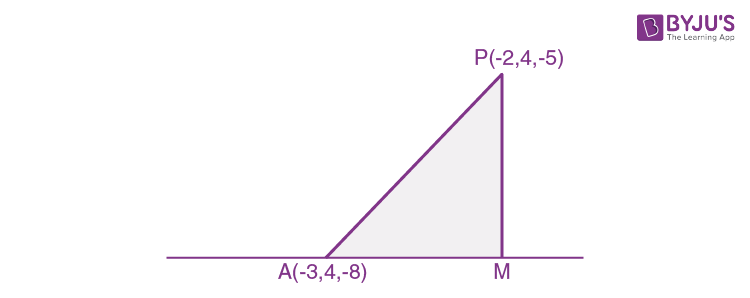Therefore, AM is equal to the projection of AP on b

AM = |AP . b / |b||

= |[(– i – 3k) (3i + 5j + 6k)] / [|(3i + 5j + 6k)|] = |(- 3 – 18) / √9 + 25 + 36| = 21 / √70

Therefore

PM = √AP2 – AM2

= √10 – (441 / 70)

= √259 / 70

= √37 / 10

Question 55: A box has 100 pens of which 10 are defective. The probability that out of a sample of 5 pens drawn one by one with replacement and at most one is defective is –

a. 9 / 10
b. (1 / 2) (9 / 10)4

c. (9 / 10)5 + (1 / 2) (9 / 10)4

d. (1 / 2) (9 / 10)5

The probability of the defective ball is, P = 10 / 100 = 1 / 10

The probability that the ball is not defective is,

Q = 1 – (1 / 10) = 9 / 10

The probability the out of a sample of 5 pens drawn one by one without replacement and almost one is defective calculated as,

Probability = 5C0 (1 / 10)0 (9 / 10)5 + 5C1 (1 / 10)1 (9 / 10)4

= (1) (9 /10)5 + (5 / 10) (9 / 10)4

= (9 / 10)5 + (1 / 2) (9 / 10)4

Question 56: Two events A and B will be independent if –

a. A and B are mutually exclusive
b. P (A’ ∩ B’) = (1 – P (A)) (1 – P (B))
c. P (A) = P (B)
d. P (A) + P (B) = 1

Two events A & B are said to be independent if,

P (A’ ∩ B’) = P (A’) . P (B’)

If A and B are independent then A’ and B’ are also independent. Therefore,

P (A’ ∩ B’) = P (A’) . P (B’) = (1 – P (A)) (1 – P (B))

If A and B are mutually exclusive then, P (A ∩ B) = 0

Check the options.

Question 57: The probability distribution of X is

 X 0 1 2 3 P (X) 0.3 k 2k 2k

The value of k is –

a. 0.14
b. 0.3
c. 0.7
d. 1

The sum of all probabilities is always equal to 1.

So,

0.3 + K + 2K + 2K = 1

0.3 + 5K = 1

5K = 0.7

K = 0.7 / 5

K = 0.14

Question 58: The shaded region in the figure is the solution set of the in equations –a. 5x + 4y ≥ 20, x ≤ 6, y ≥ 3, x ≥ 0, y ≥ 0
b. 5x + 4y ≤ 20, x ≤ 6, y ≤ 3, x ≥ 0, y ≥ 0
c. 5x + 4y ≥ 20, x ≤ 6, y ≤ 3, x ≥ 0, y ≥ 0
d. 5x + 4y ≥ 20, x ≥ 6, y ≤ 3, x ≥ 0, y ≥ 0

(1) Since, the region (shaded) lies above the x-axis.

y ≥ 0

(2) Shaded region is on left of x = 6

x ≤ 6

(3) Shaded region is below y = 3 y 3 (4)

Equation of line passing through (4, 0) & (0, 5) is

y – 0 = [5 / – 4] (x – 4)

5x + 4y – 20 = 0

If 5x + 4y ≥ 20, put (0, 0) we get 0 ≥ 20

False

Region lies away from origin which is true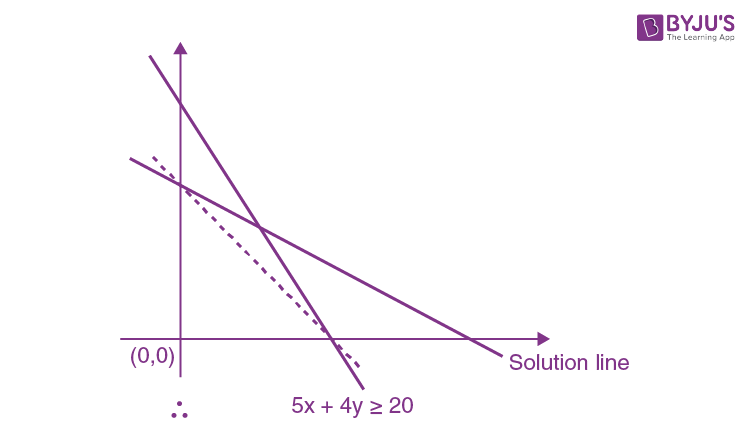(5) Shaded region lies on right side of y-axis

x ≥ 0

5x + 4y ≥ 20, x ≤ 6, y ≤ 3, x ≥ 0, y ≥ 0

Question 59: If an LPP admits an optimal solution at two consecutive vertices of a feasible region, then –

a. The required optimal solution is at the midpoint of the line joining two points
b. The optimal solution occurs at every point on the line joining these two points
c. The LPP under consideration is not solvable
d. The LPP under consideration must be reconstructed

When a LPP admits an optimal solution at two consecutive vertices of a feasible region. Then the optimal solution occurs at every point on the line joining these two points.

Question 60: 0.23.5 [x] dx is equal to:

a. 4
b. 4.5
c. 3.5
d. 3

$$\begin{array}{l}\\I=\int_{0.2}^{3.5}[x]dx\\ =\int_{0.2}^{1}[x]dx+\int_{1}^{2}[x]dx+\int_{2}^{3}[x]dx+\int_{3}^{3.5}[x]dx\\ =\int_{0.2}^{1}0\ dx+\int_{1}^{2}1 \ dx+\int_{2}^{3}2 \ dx+\int_{3}^{3.5}3 \ dx\\ =0+[x]_{1}^{2}+2[x]_{2}^{3}+3[x]_{3}^{3.5}\\ = (2 – 1) + 2(3- 2) + 3(3.5 – 3)\\ =1 + 2 + 1.5\\ I=4.5\end{array}$$